# Axiomatic Theories of Partial Ground II

Partial Ground and Hierarchies of Typed Truth

## Abstract

This is part two of a two-part paper in which we develop an axiomatic theory of the relation of partial ground. The main novelty of the paper is the of use of a binary ground predicate rather than an operator to formalize ground. In this part of the paper, we extend the base theory of the first part of the paper with hierarchically typed truth-predicates and principles about the interaction of partial ground and truth. We show that our theory is a proof-theoretically conservative extension of the ramified theory of positive truth up to 𝜖 0 and thus is consistent. We argue that this theory provides a natural solution to Fine’s “puzzle of ground” about the interaction of truth and ground. Finally, we show that if we apply the truth-predicate to sentences involving our ground-predicate, we run into paradoxes similar to the semantic paradoxes: we get ground-theoretical paradoxes of self-reference.

## Introduction

This is part two of a two-part paper in which we develop axiomatic theories of partial ground.Footnote 1 Partial ground, remember, is the relation of one truth holding (either wholly or partially) in virtue of another, cf. [5, 7].Footnote 2 Partial ground in this sense is standardly taken to be irreflexive, meaning that no truth partially grounds itself, and transitive, meaning that partial grounds are inherited through partial grounding. In other words, partial ground is a strict partial order on the truths.Footnote 3 Consequently, the relation of partial ground induces a hierarchy of grounds on the truths, in which the partial grounds of a truth rank “strictly belon the truth itself. In the first part of the paper, we axiomatized this hierarchy over the truths of arithmetic using a (binary) ground predicate instead of an operator to formalize the relation of partial ground.Footnote 4

When axiomatizing the grounding hierarchy, we explicitly restricted ourselves to applications of the ground predicate to arithmetical truths (i.e. true sentences in the language of arithmetic), leaving aside applications of the ground predicate to sentences involving the truth predicate and applications of the truth predicate to sentences involving the ground predicate. In the present paper, we lift these restrictions.

First, we turn our attention to applications of the ground predicate to sentences involving the truth predicate. A plausible principle about the interaction of truth and partial ground states that the truth of any true sentence grounds the truth of the sentence which states that the sentence in question is true. This principle traces back to Aristotle and may be put a bit more explicitly as follows:

• (Aristotelian Principle): If φ is a true sentence, then the truth of $$Tr(\ulcorner \varphi \urcorner )$$ holds either wholly or partially in virtue of the truth of φ.Footnote 5

Unfortunately, as Fine effectively shows in , the Aristotelian Principle is inconsistent with standardly accepted principles about the interaction of partial ground and the logical operators: it allows us to derive that the truths of some true sentences partially ground themselves—in direct contradiction to the irreflexivity of partial ground. To give a quick example, take the sentence ∃x T r(x), which says that there is at least one true sentence. This sentence is itself (provably) true, and so we have that ∃x T r(x) partially grounds $$Tr(\ulcorner \exists xTr(x)\urcorner )$$ by the Aristotelian Principle. By the standardly accepted principle of partial ground that an existential truth is partially grounded in all of its true instances, we get furthermore that $$Tr(\ulcorner \exists xTr(x)\urcorner )$$ partially grounds ∃x T r(x). But by the transitivity of partial ground this immediately gives us that ∃x T r(x) partially grounds itself. This is (an instance of) what in  Fine calls the puzzle of ground.

In this paper, we develop, in quite some formal detail, what Fine [6, p. 108–10] calls a “predicativist” solution to the puzzle of ground. What makes our solution predicativist is our appeal to Tarski’s hierarchy of object-language, meta-language, meta-meta-language, and so on to rule out the problematic cases of apparently self-grounding sentences. We show that by observing Tarski’s distinction between object and meta-language, we can formulate a consistent axiomatic theory of partial ground that proves (a predicativist version of) the Aristotelian principle while retaining the irreflexivity of ground. Formally, we obtain this theory using the method typing, familiar from theories of truth, where applications of a truth-predicate to sentences involving the same truth-predicate are ruled out.Footnote 6 To the best of our knowledge, this is the first fully worked out proposal for a predicativist solution to the puzzle of ground in the literature.Footnote 7

Then, we turn our attention to applications of the truth predicate to sentences containing the ground predicate. We show that we cannot consistently add axioms to our axiomatic theory of ground that would allow us to prove all the instances of the T-scheme $$Tr(\ulcorner \varphi \urcorner )\leftrightarrow \varphi$$ over formulas φ involving the ground-predicate; for if we were do so, we’d get what we call ground-theoretic paradoxes of self-reference. We will show that these paradoxes are closely related to the well-known semantic paradoxes of self-reference, such as the infamous liar paradox, and furthermore that the paradoxes are genuinely different from Fine’s puzzle of ground. Fine’s puzzle arises from the fact that intuitively plausible principles about partial ground and truth entail that the truths of some sentences partially ground themselves. The ground-theoretic paradoxes of self-reference, in contrast, concern an entirely different aspect of partial ground: the problem is, as we’ll argue, that the ground predicate behaves too much like a truth-predicate.Footnote 8

Here is the plan for the paper: Section 1.1 contains a précis of the first part of the paper. Then, in Section 2, we’ll discuss the interaction of truth and partial ground on a general level and introduce the Aristotelian Principle into our predicational setting for axiomatic theories of partial ground. In Section 3, we’ll develop an axiomatic predicational theory that proves (a predicative/typed version of) the Aristotelian principle. We then prove that the theory is a conservative extension of the ramified theory of truth (and is thus, in particular, consistent) and we construct a model. Then, in Section 4, we will show that if we’re not careful, partial ground, just like truth, can give rise to paradoxes of self-reference. In Section 5, we conclude with some general observations and a map of possible responses to the ground-theoretic paradoxes of self-reference.

### Précis of “Axiomatic Theories of Partial Ground I. The Base Theory”

In the following sections, we assume that the reader is familiar with the techniques and results obtained in the first part of the paper. Those who have these techniques and results sufficiently present in their mind can safely skip this section. But for the rest, let’s briefly refresh our memory.

In the first part of the paper, we develop an axiomatic theory of partial ground using a binary ground predicate rather than an operator to express partial ground. We call such a theory a predicational theory of partial ground, in contrast to operational theories. At the outset of the paper, we provide technical and philosophical reasons for using a predicational approach (Section 2).

In the following sections (Sections 3 and 4), we develop our theory in formal detail and investigated its properties. On the syntax side, we start from the language $$\mathcal {L}$$ of Peano arithmetic. The language $$\mathcal {L}_{Tr}$$ is defined as $$\mathcal {L}\cup \{Tr\}$$, where T r is the unary truth-predicate, and the language $$\mathcal {L}_{Tr}^{\lhd }$$ is $$\mathcal {L}_{Tr}\cup \{\lhd \}$$, where $$\lhd$$ is the binary (partial) ground-predicate. We formulate our theory in $$\mathcal {L}_{Tr}^{\lhd }$$.

On the theory side, we starte from the theory PAG of Peano arithmetic (PA) with all the instances of the induction scheme

$$\varphi({0})\land \forall x(\varphi(x)\to \varphi(Sx))\to \forall x\varphi(x)$$

over the language $$\mathcal {L}_{Tr}^{\lhd }$$. To formulate our predicational theory of ground, we additionally rely on standard syntax theory developed in PA. In particular, we use a standard coding function # to map every expression (variable, term, formula, etc.) σ to a unique code # σ from the natural numbers. If σ is an expression, then we also write $$\ulcorner \sigma \urcorner$$ for the numeral intended to denote # σ. We use function terms ¬̣, ∧̣, ∧̣, ∃̣, ∀̣, = ̣ and $$\lhd$$̣ to represent the corresponding syntactic operations.Footnote 9

Now our predicational theory of ground PG has all the axioms of PAG, plus the following axioms:Footnote 10

Basic Ground Axioms:                                  Basic Truth Axioms:

• G1 $$\forall x\neg (x\lhd x)$$                                  T1st(T r(s$$t)\leftrightarrow s^{\circ }=t^{\circ })$$

• G2 $$\forall x\forall y\forall z(x\lhd y\land y\lhd z\to x\lhd z)$$                                  T$$_{2} \forall s\forall t(Tr(s\underset {.}{\neq }t)\leftrightarrow s^{\circ }\neq t^{\circ })$$

• G3 $$\forall x\forall y(x\lhd y\to Tr(x)\land Tr(y))$$                                   T3x(T r(x) → S e n t(x))

• Upward Directed Axioms:

U1 $$\forall x (Tr(x)\to x\lhd \underset {.}{\neg }\underset {.}{\neg }x)$$

• U2 $$\forall x\forall y ((Tr(x)\to x\lhd x\underset {.}{\lor }y) \land (Tr(y)\to y\lhd x\underset {.}{\lor }y))$$

• U3 $$\forall x\forall y (Tr(x)\land Tr(y)\to (x\lhd x\underset {.}{\land } y)\land (y\lhd x\underset {.}{\land } y))$$

• U4 $$\forall x\forall y (Tr(\underset {.}{\neg } x)\land Tr(\underset {.}{\neg } y)\to (\underset {.}{\neg } x\lhd \underset {.}{\neg }(x\underset {.}{\lor } y))\land (\underset {.}{\neg } y\lhd \underset {.}{\neg }(x\underset {.}{\lor } y)))$$

• U5 $$\forall x\forall y ((Tr(\underset {.}{\neg }x)\to \underset {.}{\neg }x\lhd \underset {.}{\neg }(x\underset {.}{\land }y)) \land (Tr(\underset {.}{\neg }y)\to \underset {.}{\neg }y\lhd \underset {.}{\neg }(x\underset {.}{\land }y)))$$

• U6 $$\forall x \forall t\forall v(Tr(x(t/v))\to x(t/v)\lhd \underset {.}{\exists } v x)$$

• U7 $$\forall x\forall v (\forall tTr(\underset {.}{\neg } x(t/v))\to \forall t(\underset {.}{\neg }x(t/v)\lhd \underset {.}{\neg }\underset {.}{\exists }v x))$$

• U8 $$\forall x \forall v(\forall t(Tr(x(t/v))\to \forall t(x(t/v)\lhd \underset {.}{\forall }v x))$$

• U9 $$\forall x \forall t\forall v(Tr(\underset {.}{\neg }x(t/v))\to \underset {.}{\neg }x(t/v)\lhd \underset {.}{\neg }\underset {.}{\forall } v x))$$

• Downward Directed Axioms:

D 1 $$\forall x(Tr(\underset {.}{\neg }\underset {.}{\neg }x)\to x\lhd \underset {.}{\neg }\underset {.}{\neg }x)$$

• D 2 $$\forall x\forall y (Tr(x\underset {.}{\lor }y)\to (Tr(x)\to x\lhd x\underset {.}{\lor }y )\land (Tr(y)\to y\lhd x\underset {.}{\lor }y))$$

• D 3 $$\forall x\forall y (Tr(x\underset {.}{\land }y)\to (x\lhd x\underset {.}{\land } y)\land (y\lhd x\underset {.}{\land } y))$$

• D 4 $$\forall x\forall y (Tr(\underset {.}{\neg } (x\underset {.}{\land } y))\to (Tr(\underset {.}{\neg }x)\to \underset {.}{\neg } x\lhd \underset {.}{\neg }(x\underset {.}{\lor } y))\land (Tr(\underset {.}{\neg }y)\to \underset {.}{\neg } y\lhd \underset {.}{\neg }(x\underset {.}{\lor } y)))$$

• D 5 $$\forall x\forall y (Tr(\underset {.}{\neg } (x\underset {.}{\lor } y))\to (\underset {.}{\neg } x\lhd \underset {.}{\neg }(x\underset {.}{\lor } y))\land (\underset {.}{\neg } y\lhd \underset {.}{\neg }(x\underset {.}{\lor } y)))$$

• D 6 $$\forall x (Tr(\underset {.}{\exists } v x(v))\to \exists t(x(t/v)\lhd \underset {.}{\exists } v x))$$

• D 7 $$\forall x \forall v(Tr(\underset {.}{\neg }\underset {.}{\exists }v x)\to \forall t(\underset {.}{\neg }x(t/v)\lhd \underset {.}{\neg }\underset {.}{\exists }v x))$$

• D 8 $$\forall x \forall v(Tr(\underset {.}{\forall }v x\to \forall t(x(t/v)\lhd \underset {.}{\forall }v x))$$

• D 9 $$\forall x \forall v(Tr(\underset {.}{\neg }\underset {.}{\forall } v x)\to \exists t(\underset {.}{\neg }x(t/v)\lhd \underset {.}{\neg }\underset {.}{\forall } v x))$$

In the remaining sections of part one of the paper, we show that PG is a proof-theoretically conservative extension of the theory PT of positive truth (Theorem 1) and provide a model construction (Theorem 2) by extending the standard model of PT to a canonical model of PG. As an immediate corollary of the conservativity result, we infer that PG is consistent (Corollary 3) and that PG he same arithmetic statements as the theory ACA of arithmetic comprehension (Corollary 4).

## The Aristotelian Principle and Typed Truth

Aristotle has the following to say about the interaction of truth and explanation:

It is not because we think truly that you are pale, that you are pale; but because you are pale we who say this have the truth.

(Metaphysics 1051b6–9)

In the context of partial ground, this motivates the following principle: If φ is true, then it is natural to say that φ is true in virtue of what it says being the case, and if φ is false, then φ is false in virtue of what it says not being the case. We will thus call the corresponding informal ground-theoretic principles the Aristotelian principles about truth and falsehood respectively.

If we wish to formalize the Aristotelian principles in the context of our predicational theory of ground, we have to make a couple of adjustments. First, we have to assume that we have a Gödel-numbering for the language $$\mathcal {L}_{Tr}$$. In particular, we now assume that we have a name $$\ulcorner \varphi \urcorner$$ for every sentence $$\varphi \in \mathcal {L}_{Tr}$$. Second, we have to adjust our basic truth axioms. The axioms T 3 and G 3 of PG together ensure that both the truth predicate and the ground predicate only apply to sentences of $$\mathcal {L}$$. To formalize the Aristotelian principles, however, we need to relax this requirement: we need for the truth predicate and the ground predicate to apply to sentences of $$\mathcal {L}_{Tr}$$ that are not already in $$\mathcal {L}$$. To allow for this, we adjust the axiom T 3 to the axiom:

$$\forall x(Tr(x)\to Sent_{Tr}(x)),$$

which we’ll label T$$_{3}^{\ast }$$. Together with G 3, this then entails:

$$\forall x\forall y(x\lhd y\to Sent_{Tr}(x)\land Sent_{Tr}(y)),$$

as well. We’ll refer to the theory that results from replacing T3 in PG with T$$_{3}^{\ast }$$ as PGT. Thus, in PGT, we are not only talking about the truths of arithmetic, but also about the truth of the truths of arithmetic.

With these adjustments in place, we can schematically express the Aristotelian principle about truth by saying that for all sentences φ that:

$$\varphi\to \ulcorner\varphi\urcorner\lhd \ulcorner Tr(\ulcorner\varphi\urcorner)\urcorner.$$

For the Aristotelian principle about falsehood, we get:

$$\neg\varphi\to \ulcorner\neg \varphi\urcorner\lhd \ulcorner \neg Tr(\ulcorner\varphi\urcorner)\urcorner,$$

for all sentences φ. Using the same strategy as in the previous paper, we can translate these schemata into the quantified axioms:

• (AP T ) $$\forall x(Tr(x)\to x\lhd \underset {.}{Tr}(\dot {x})),$$ and

• (AP F ) $$\forall x(Tr(\underset {.}{\neg }x)\to \underset {.}{\neg }x\lhd \underset {.}{\neg }\underset {.}{Tr}(\dot {x})),$$

where $$\underset {.}{Tr}$$ represents the function that maps the code # t of a term t to the code # T r(t) of the application T r(t) of the truth predicate to the term t. Thus, we have arrived at a quantified axiomatization of the Aristotelian principles.Footnote 11

Unfortunately, as  shows, the Aristotelian principles are ground-theoretically inconsistent:Footnote 12

### Lemma 1 (Puzzle of Ground)

A P T and A P F are inconsistent over PGT.

### Proof

To show that $$AP_{T}\vdash _{PGT}\bot$$ consider the following derivation:

1. 1.

$$\overline {0}=\overline {0}$$ (Arithmetic)

2. 2.

$$Tr(\ulcorner \overline {0}=\overline {0}\urcorner )$$ (T-scheme over $$\mathcal {L}$$)

3. 3.

$$\ulcorner \overline {0}=\overline {0}\urcorner \lhd \ulcorner Tr(\ulcorner \overline {0}=\overline {0}\urcorner )\urcorner$$ (2., AP T )

4. 4.

$$Tr(\ulcorner Tr(\ulcorner \overline {0}=\overline {0}\urcorner )\urcorner )$$ (3., G 3)

5. 5.

$$\ulcorner Tr(\ulcorner \overline {0}=\overline {0}\urcorner )\urcorner \lhd \exists x Tr(x)$$ (4., U 6)

6. 6.

$$Tr(\ulcorner \exists x Tr(x)\urcorner )$$ (5., G 3)

7. 7.

$$\ulcorner \exists x Tr(x)\urcorner \lhd \ulcorner Tr(\ulcorner \exists x Tr(x)\urcorner )\urcorner$$ (6., AP T )

8. 8.

$$Tr(\ulcorner Tr(\ulcorner \exists x Tr(x)\urcorner )\urcorner )$$ (7., G 3)

9. 9.

$$\ulcorner Tr(\ulcorner \exists x Tr(x)\urcorner )\urcorner \lhd \ulcorner \exists x Tr(x)\urcorner$$ (8., U 6)

10. 10.

$$\ulcorner \exists x Tr(x)\urcorner \lhd \ulcorner \exists x Tr(x)\urcorner$$ (7.,9., G 2)

11. 11.

$$\neg (\ulcorner \exists x Tr(x)\urcorner \lhd \ulcorner \exists x Tr(x)\urcorner )$$ (G 1)

12. 12.

⊥ (10.,11., ⊥-Intro)

To show that $$AP_{F}\vdash _{PGT}\bot ,$$ we can perform an analogous derivation, which is left to the interested reader. □

We are left with a ground-theoretic puzzle about truth.Footnote 13 All the principles involved in the proof of Lemma 1 are intuitively plausible: the basic ground axioms G 2 and G 3 directly arise from the definition of partial ground, the upward directed axiom U 6 about the existential quantifier is plausible in light of the usual semantics for first-order logic, and the Aristotelian principle for truth is plausible from considerations about truth. (The principles required to show that AP F is ground-theoretically inconsistent are equally plausible.) So what are we to do? In this section, we will propose a solution to the puzzle of ground that preserves the intuition behind all of these principles. We will achieve this by typing our truth predicate—a move familiar from typed theories of truth.

In typed theories of truth, no applications of the truth predicate to sentences containing the same truth predicate are provable. Thus, typed theories of truth respect Tarski’s distinction between object-language and meta-language . Tarski motivates this distinction from the semantic paradoxes, such as the infamous liar paradox. This paradox results when we apply the T-scheme to the liar sentence which intuitively “says of itself” that it is not true. More specifically, Tarski observed that, in a sufficiently strong background theory, such as PA, if we allow applications of the truth predicate to sentences with the same truth predicate in them, we get a sentence λ that is provably equivalent to its own falsehood:

$$\vdash_{PA}\lambda \leftrightarrow \neg Tr(\ulcorner \lambda\urcorner).$$

This follows from the so-called diagonal lemma, which is provable in PA in the context of an appropriate Gödel-numbering for $$\mathcal {L}_{Tr}$$:Footnote 14

### Lemma 2

For every sentence $$\varphi (x)\in \mathcal {L}_{Tr}$$ with exactly one free variable x,there is a sentence $$\delta \in \mathcal {L}_{Tr}$$ such that

$$\vdash_{PA} \delta\leftrightarrow \varphi(\ulcorner\delta\urcorner).$$

The existence of the liar sentence λ, then, follows by a simple application of the diagonal lemma to the formula $$\neg Tr(x)\in \mathcal {L}_{Tr}$$. It is well-known that the existence of a liar sentence is inconsistent with the T-scheme over the language $$\mathcal {L}_{Tr}$$.

A common intuitive response to the liar paradox is that it somehow arises from the self-reference involved.Footnote 15 On this informal view, the problem is that the liar sentence “says something of itself,” namely that it is not true.Footnote 16 Thus, so the intuitive response, we should put restrictions on our language that prevent self-reference. Tarski makes this response precise by introducing the distinction between object-language and meta-language. To illustrate the distinction, consider the truths of arithmetic. According to Tarski, if we wish to talk about numbers and their properties, we can do so in the language $$\mathcal {L}$$ of PA—our object-language for arithmetic. But if we wish talk about the truth of sentences in $$\mathcal {L}$$, we have to do so in the language $$\mathcal {L}_{Tr}$$—our meta-language for the truths of arithmetic.Footnote 17 Moreover, if we wish to talk about the truth of the truths of arithmetic, i.e. the truths of sentences in $$\mathcal {L}_{Tr}$$ containing the truth predicate, we need to do so in yet another meta- meta-language, which has a distinct truth-predicate for the sentences of $$\mathcal {L}_{Tr}$$. And so on. In contrast, Tarski calls a language that can talk about the truths of its own sentences, i.e. a language that has both names for all of its sentences and a truth predicate that applies to these names, semantically closed. Thus, a semantically closed language is its own meta-language, as it were, and thus we get self-referential paradoxes. Tarski shows that if we obey the distinction between object-language and meta-language, we can formulate a consistent theory of truth: In an appropriate meta-language, which is not semantically closed, we can consistently affirm the T-scheme for the sentences of the object-language and we never can prove problematic sentences, such as the liar. The liar paradox, on the other hand, shows that if we work in a semantically closed language, disaster ensues: If we have a Gödel-numbering for the terms of $$\mathcal {L}_{Tr}$$ within $$\mathcal {L}_{Tr}$$ and at the same time affirm the T-scheme over the sentences of $$\mathcal {L}_{Tr},$$ i.e. if we use $$\mathcal {L}_{Tr}$$ as its own meta-language, we get semantic paradoxes, like the liar paradox. Thus, so Tarski argues, when we wish to talk about truth, we should not never do so in a semantically closed language, but always in an appropriate meta-language. Intuitively, the picture is that semantic truths, such as truths about the truths of arithmetic, are on a “higher level” than non-semantic truths, such as the ordinary truths of arithmetic. Moreover, this can be iterated: the truths about truths about the truths of arithmetic are on yet a “higher level” than the truths about the truths of arithmetic and so on. What emerges is Tarski’s hierarchy of truths. Following Tarski, if we work in a semantically closed language, we mix the levels of the hierarchy of truths—and ultimately this is the source of the semantic paradoxes.Footnote 18

Our original, unmodified predicational theory of ground PG respects Tarski’s distinction between object- and meta-language: We have formulated PG in the language $$\mathcal {L}_{Tr}^{\lhd }$$ in the context of a coding for the language $$\mathcal {L}$$ of PA. In particular, we have assumed that we have a name $$\ulcorner \varphi \urcorner$$ for every sentence $$\varphi \in \mathcal {L},$$ but not that we have names $$\ulcorner Tr(t)\urcorner$$ for sentences of the form $$Tr(t)\in \mathcal {L}_{Tr}$$ and so on. Moreover, as we have said before, by the axioms T 3 and G 3, we have ensured that both the truth predicate and the ground predicate only apply to the sentences of $$\mathcal {L}$$. Thus, we have used the language $$\mathcal {L}_{Tr}^{\lhd }$$ as an appropriate meta-language for our object-language $$\mathcal {L}$$—in compliance with Tarski’s distinction. When we move to the modified theory P G T, however, we no longer conform with Tarski’s distinction. Since PGT is formulated in $$\mathcal {L}_{Tr}^{\lhd }$$ in the context of a Gödel numbering for $$\mathcal {L}_{Tr}$$, PGT is formulated in a semantically closed language. Now, the truth predicate may apply to sentences with the same truth predicate in them: Since in PGT we work in the context of a coding for $$\mathcal {L}_{Tr},$$ we have names for all the sentences of $$\mathcal {L}_{Tr}^{\lhd }$$ within $$\mathcal {L}_{Tr}^{\lhd }$$ itself. Moreover, by the axiom T$$_{3}^{\ast }$$ we have allowed for these terms to occur truly in the context of the truth predicate and the ground predicate. In other words, when we formulated P G T, we have used $$\mathcal {L}_{Tr}^{\lhd }$$ as its own meta-language—we talked about the truths of $$\mathcal {L}_{Tr}^{\lhd }$$ within $$\mathcal {L}_{Tr}^{\lhd }$$ itself.

Based on this observation, we argue that the semantic closure of $$\mathcal {L}_{Tr}^{\lhd }$$ is (at least part of) the reason for why the puzzle of ground arises. Note that the semantic closure of $$\mathcal {L}_{Tr}^{\lhd }$$ is required for the proof of Lemma 1. In the third step of the derivation, we applied the ground predicate to the truth predicate in $$\ulcorner \overline {0}=\overline {0}\urcorner \lhd \ulcorner Tr(\ulcorner \overline {0}=\overline {0}\urcorner )\urcorner .$$ Moreover, in the fourth step, we inferred $$Tr(\ulcorner Tr(\ulcorner \overline {0}=\overline {0}\urcorner )\urcorner )$$ from this and thus applied the truth predicate to a sentence containing the same truth predicate. The main difference between the liar paradox and the puzzle of ground is that, in the case of the liar, we get a truth-theoretic inconsistency, i.e. an inconsistency with plausible principles for truth, while in the case of the puzzle, we get a ground-theoretic inconsistency, i.e. an inconsistency with plausible principles for partial ground. Still, the problematic sentences in both cases are quite similar. In both cases some intuitive form of self-reference is involved: while the liar sentence λ intuitively says something of itself, the principles of partial ground entail that truth of ∃x T r(x) partially grounds itself. Thus, we can say that the self-reference in the case of the liar is semantic, while the self-reference in the case of the puzzle of ground is ground-theoretic.Footnote 19

In analogy to typed theories of truth, we propose a typed solution to the puzzle of ground. To formulate this solution, we will move to a slightly different framework, where instead of a single truth predicate T r, we have a family T r 1,T r 2,… of typed truth predicates. These truth predicates intuitively express truth on the first, second, … level of Tarski’s hierarchy. In the remainder of this section, we will develop a consistent theory of partial ground and typed truth using these typed truth predicates. This theory will contain typed versions of the axioms of PG plus typed versions of the Aristotelian principles. Much like in the case of typed theories of truth, this will mean that no sentence is provable in which the truth predicate is applied to a sentence containing the same truth predicate. We will show that this restriction is sufficient to obtain a consistent theory of partial ground and typed truth.

## Axiomatic Theories of Partial Ground and Typed Truth

Typed theories of truth aim to axiomatize Tarski’s hierarchy of truths.Footnote 20 For this purpose, in typed theories of truth, we have different truth predicates for the different levels of the hierarchy. Correspondingly, we get a hierarchy of languages with a different language for every level of the hierarchy. To illustrate, we start with $$\mathcal {L}_{0}=_{def}\mathcal {L}$$—the language of PA. The truth predicate for sentences of arithmetic is, then, T r 1 and the language $$\mathcal {L}_{1}$$ extends $$\mathcal {L}_{0}$$ with T r 1. The truth predicate for sentences of $$\mathcal {L}_{1}$$, in turn, is T r 2 and the language $$\mathcal {L}_{2}$$ extends $$\mathcal {L}_{1}$$ with T r 2. And so on. Thus, typed theories of truth are formulated using a hierarchical family of truth predicates T r 1,T r 2,… that intuitively correspond to truth on the different levels of Tarski’s hierarchy.

### Language and Background Theory

We will now formally define a hierarchy of languages, such that on every level we can talk about the truth of sentences on the lower levels. For reasons of generality, we will define this hierarchy in such a way that it includes even infinitary levels. Specifically, we assume that for every ordinal 0 < α < 𝜖 0, we have a different truth predicate T α that intuitively expresses truth at the level α: we have $$Tr_{1}, \mathellipsis , Tr_{\omega }, \mathellipsis , Tr_{\omega ^{\omega }},\mathellipsis , Tr_{\omega ^{\omega ^{\omega }}}, \mathellipsis ,$$ where for αβ < 𝜖 0, we have T r α T r β .Footnote 21 For all ordinals 1 ≤ α𝜖 0, we define the language $$\mathcal {L}_{<\alpha }$$ as the language $$\mathcal {L}$$ of PA extended with all the truth predicates T β for 0 < β < α:

$$\mathcal{L}_{<\alpha}=_{def}\mathcal{L}\cup\{Tr_{\beta}~|~0<\beta<\alpha\}.$$

Then we set:

$$\mathcal{L}_{\alpha}=_{def} \mathcal{L}_{<\alpha+1},$$

for all ordinals 0 ≤ α < 𝜖 0. Thus, the language $$\mathcal {L}_{0}$$ is $$\mathcal {L},$$ the language $$\mathcal {L}_{1}$$ is $$\mathcal {L}\cup \{Tr_{1}\}$$, and so on. Intuitively, for an ordinal 1 ≤ α𝜖 0, the language $$\mathcal {L}_{<\alpha }$$ talks about the truths at the levels strictly below α and $$\mathcal {L}_{\alpha }$$ talks about the truths at all levels up to and including α. When we are operating on the ordinal level α, the language $$\mathcal {L}_{<\alpha }$$ is our intended object-language, i.e. we wish to talk about grounding between the truths of sentences in $$\mathcal {L}_{<\alpha }$$. For most informal purposes, however, we already stop at the level of $$\mathcal {L}_{<2}=\mathcal {L}\cup \{Tr_{1}\}$$. The reason for this is that $$\mathcal {L}_{<2}$$ is the first language in which grounding between arithmetic truths and truths involving a truth predicate occurs. For all ordinals 1 ≤ α𝜖 0, the language $$\mathcal {L}_{<\alpha }^{\lhd }$$ is $$\mathcal {L}_{<\alpha }$$ extended with our binary ground predicate $$\lhd$$:

$$\mathcal{L}_{<\alpha}^{\lhd}=_{def}\mathcal{L}_{<\alpha}\cup\{\lhd\}.$$

And we set:

$$\mathcal{L}_{\alpha}^{\lhd}=_{def}\mathcal{L}_{\alpha}\cup\{\lhd\},$$

for all ordinals 0 ≤ α < 𝜖 0. When we are operating on the ordinal level α, we’ll use $$\mathcal {L}_{\alpha }^{\lhd }$$ as our meta-language for the object-language language $$\mathcal {L}_{<\alpha }$$. Again, for expository purposes, we’ll usually stop at $$\mathcal {L}_{2}^{\lhd }=\mathcal {L}_{2}\cup \{\lhd \},$$ which is the first language in which we can talk about grounding in $$\mathcal {L}_{<2}=\mathcal {L}_{1}$$.

For an ordinal 0 < α < 𝜖 0, the theory P A T <α is the result of extending PA with all the instances of the induction scheme over the language $$\mathcal {L}_{<\alpha }$$ and the theory P A G <α is the result of extending P A T <α with all the missing instances of the induction scheme over $$\mathcal {L}_{\alpha }^{\lhd }$$. For 0 ≤ α < 𝜖 0, the theory P A T α , then, is P A T <α+1 and similarly P A G α is P A G <α+1. Thus, P A T 0 is PAT and P A G 0 is PAG. In P A T α , we can develop a syntax theory for the languages $$\mathcal {L}_{<\alpha }$$ analogously to the way we developed the syntax theory in the first part of this paper. When we work on an ordinal level α, we assume that in $$\mathcal {L}_{\alpha }^{\lhd },$$ via some appropriate Gödel coding, we have names $$\ulcorner \varphi \urcorner$$ for all formulas $$\varphi \in \mathcal {L}_{<\alpha }$$.Footnote 22 Moreover, we assume that for every 0 < β < α, we have a function symbol $$\underset {.}{Tr_{\beta }}$$ that represents the function which maps the code # t of a term t to the code # T r β (t) of the formula $$Tr_{\beta }(t)\in \mathcal {L}_{<\alpha }$$. And we abbreviate the formula that allows us to (strongly) represent the (set of codes of) sentences in $$\mathcal {L}_{<\alpha }$$ by S e n t <α .

### Axioms for Partial Ground and Typed Truth

With the syntax in place, we extend our theory PG to account for partial ground between truths on the same level of Tarski’s hierarchy. We’ll define this extension from the perspective of some ordinal level 0 < α < 𝜖 0. Thus, we wish to talk about ground between truths on all the ordinal levels 0 < β < α. To achieve this, we have to modify the basic ground axiom G 3 and the basic truth axioms T 1, T 2, and T 3. The axiom G 3 splits up in the following pair, for all ordinals 0 < β < α:

• (G$$_{3a}^{\beta }$$) $$\forall x\forall y (x\lhd y\to (Sent_{<\beta }(x)\to Tr_{\beta }(x)))$$

• (G$$_{3b}^{\beta }$$) $$\forall x\forall y (x\lhd y\to (Sent_{<\beta }(y)\to Tr_{\beta }(y)))$$

These axioms formalize the factivity of ground in a typed context. In particular, the axiom G$$_{3a}^{\beta }$$ says that if the truth of some sentence grounds the truth of another, and if the sentence is below the level β in the hierarchy, then it is true at level β of the hierarchy. The axiom G$$_{3b}^{\beta },$$ on the other hand, says the same thing the other way around: if the truth of some sentence is grounded in the truth of another, and if the former sentence is below level β, then it is true at level β. To illustrate, if we let α = 2, we get the following axiom pair:

• (G$$_{3a}^{1}$$) $$\forall x\forall y(x\lhd y\to (Sent_{<1}(x)\to Tr_{1}(x)))$$

• (G$$_{3b}^{1}$$) $$\forall x\forall y(x\lhd y\to (Sent_{<1}(y)\to Tr_{1}(y)))$$

Thus, for sentences $$\varphi ,\psi \in \mathcal {L}_{<1},$$ the axioms G$$_{3a}^{1}$$ and G$$_{3b}^{1}$$ together say that the truth of φ can only ground the truth of ψ, if φ and ψ are both true at level one—i.e. if they are truths of arithmetic.

Next, in the typed versions of T 1 and T 2, we wish to make sure that true equations are true at every level below α. Thus, for all ordinals 0 < β < α, we postulate:

• (T$$_{1}^{\beta }$$) $$\forall s\forall t(Tr_{\beta }(s\underset {.}{=}t)\leftrightarrow s^{\circ }=t^{\circ })$$

• (T$$_{2}^{\beta }$$) $$\forall s\forall t(Tr_{\beta }(s\underset {.}{\neq }t)\leftrightarrow s^{\circ }\neq t^{\circ })$$

Thus, we get for example $$Tr_{1}(\ulcorner \overline {0}=\overline {0}\urcorner ), Tr_{2}(\ulcorner \overline {0}=\overline {0}\urcorner ), \mathellipsis$$ and so on, for all ordinal levels below α.

In the case of T 3, we wish to make sure that a truth predicate T r β , for an ordinal 0 < β < α, only applies to sentences on levels below β—in compliance with Tarski’s distinction. Thus, we postulate for all 0 < β < α:

• (T$$_{3}^{\beta }$$) ∀x(T r β (x) → S e n t <β (x))

Thus, for example, we get ∀x(T r 1(x) → S e n t <1(x)), which intuitively says that the predicate T r 1 only applies to sentences of arithmetic.

Another adjustment is needed: Note that now G$$_{3a/b}^{\beta }$$ and T$$_{3}^{\beta }$$ do not entail anymore that the ground predicate applies only to sentences. To ensure this, we postulate the following final basic ground axiom:

• (G$$_{4}^{\alpha }$$) $$\forall x\forall y(x\lhd y\to Sent_{<\alpha }(x)\land Sent_{<\alpha }(y))$$

Thus, on the level α = 2, we get that $$\forall x\forall y(x\lhd y\to Sent_{<2}(x)\land Sent_{<2}(y))$$. In words: if $$x\lhd y,$$ then both x and y are sentences of $$\mathcal {L}_{<2},$$ which is just the language of arithmetic $$\mathcal {L}$$ extended with the truth predicate T r 1. Taken together, all of these modified axioms entail that our new theory respects Tarski’s distinction between object- and meta-language. Finally, we have to modify our upwards and downwards directed grounding axioms to apply on all levels below α. We achieve this by postulating that the axioms apply on all of these levels. Take the axioms U 1 and D 1 for example. They become the new typed set of axioms for all ordinals 0 < β < α:

• (U$$_{1}^{\beta }$$) $$\forall x (Tr_{\beta }(x)\to x\lhd \underset {.}{\neg }\underset {.}{\neg }x)$$

• (D$$_{1}^{\beta }$$) $$\forall x(Tr_{\beta }(\underset {.}{\neg }\underset {.}{\neg }x)\to x\lhd \underset {.}{\neg }\underset {.}{\neg }x)$$

Thus, on every level 0 < β < α, if a sentence is true on that level, then the sentence grounds its double-negation and if a double negation is true on the level, it is grounded by the sentence it is a double negation of.

Putting all of this together, we get:

### Definition 1

For all ordinals 0 ≤ α𝜖 0, the predicational theory P G <α of ground up to α, consists of the axioms of P A G <α plus the following axioms for all 0 < β < α:

Typed Ground Axioms: Typed Truth Axioms:

G1

$$\forall x\neg (x\lhd x)$$ T$$_{1}^{\beta } \forall s\forall t(Tr_{\beta }(s\underset {.}{=}t)\leftrightarrow s^{\circ }=t^{\circ })$$

G2

$$\forall x\forall y\forall z(x\lhd y\land y\lhd z\to x\lhd z)$$ T$$_{2}^{\beta } \forall s\forall t(Tr_{\beta }(s\underset {.}{\neq }t)\leftrightarrow s^{\circ }\neq t^{\circ })$$

G$$_{3a}^{\beta }$$

$$\forall x\forall y (x\lhd y\to (Sent_{<\beta }(x)\to Tr_{\beta }(x)))$$ T$$_{3}^{\beta } \forall x(Tr_{\beta }(x)\to Sent_{<\beta }(x))$$

G$$_{3b}^{\beta }$$

$$\forall x\forall y (x\lhd y\to (Sent_{<\beta }(y)\to Tr_{\beta }(y)))$$

G$$_{4}^{\alpha }$$

$$\forall x\forall y(x\lhd y\to Sent_{<\alpha }(x)\land Sent_{<\alpha }(y))$$

Typed Upward Directed Axioms:

• U$$_{1}^{\beta }$$

• $$\forall x (Tr_{\beta }(x)\to x\lhd \underset {.}{\neg }\underset {.}{\neg }x)$$

• U$$_{2}^{\beta }$$

• $$\forall x\forall y (Tr_{\beta }(x)\to x\lhd x\underset {.}{\lor }y \land Tr_{\beta }(y)\to y\lhd x\underset {.}{\lor }y)$$

• U$$_{3}^{\beta }$$

• $$\forall x\forall y (Tr_{\beta }(x)\land Tr_{\beta }(y)\to (x\lhd x\underset {.}{\land } y)\land (y\lhd x\underset {.}{\land } y))$$

• U$$_{4}^{\beta }$$

• $$\forall x\forall y (Tr_{\beta }(\underset {.}{\neg } x)\land Tr_{\beta }(\underset {.}{\neg } y)\to (\underset {.}{\neg } x\lhd \underset {.}{\neg }(x\underset {.}{\lor } y))\land (\underset {.}{\neg } y\lhd \underset {.}{\neg }(x\underset {.}{\lor } y)))$$

• U$$_{5}^{\beta }$$

• $$\forall x\forall y (Tr_{\beta }(\underset {.}{\neg }x)\to \underset {.}{\neg }x\lhd \underset {.}{\neg }(x\underset {.}{\land }y) \land Tr_{\beta }(\underset {.}{\neg }y)\to \underset {.}{\neg }y\lhd \underset {.}{\neg }(x\underset {.}{\land }y))$$

• U$$_{6}^{\beta }$$

• $$\forall x \forall t\forall v(Tr_{\beta }(x(t/v))\to x(t/v)\lhd \underset {.}{\exists } v x)$$

• U$$_{7}^{\beta }$$

• $$\forall x\forall v (\forall tTr_{\beta }(\underset {.}{\neg } x(t/v))\to \forall t(\underset {.}{\neg }x(t/v)\lhd \underset {.}{\neg }\underset {.}{\exists }v x))$$

• U$$_{8}^{\beta }$$

• $$\forall x \forall v(\forall t(Tr_{\beta }(x(t/v))\to \forall t(x(t/v)\lhd \underset {.}{\forall }v x))$$

• U$$_{9}^{\beta }$$

• $$\forall x \forall t\forall v(Tr_{\beta }(\underset {.}{\neg }x(t/v))\to \underset {.}{\neg }x(t/v)\lhd \underset {.}{\neg }\underset {.}{\forall } v x))$$

• Typed Downward Directed Axioms:

D1 $$\forall x(Tr_{\beta }(\underset {.}{\neg }\underset {.}{\neg }x)\to x\lhd \underset {.}{\neg }\underset {.}{\neg }x)$$

• D2 $$\forall x\forall y (Tr_{\beta }(x\underset {.}{\lor }y)\to (Tr_{\beta }(x)\to x\lhd x\underset {.}{\lor }y )\land (Tr_{\beta }(y)\to y\lhd x\underset {.}{\lor }y))$$

• D3 $$\forall x\forall y (Tr_{\beta }(x\underset {.}{\land }y)\to (x\lhd x\underset {.}{\land } y)\land (y\lhd x\underset {.}{\land } y))$$

• D4 $$\forall x\forall y (Tr_{\beta }(\underset {.}{\neg } (x\underset {.}{\land } y))\to (Tr_{\beta }(\underset {.}{\neg }x)\to \underset {.}{\neg } x\lhd \underset {.}{\neg }(x\underset {.}{\lor } y))\land (Tr_{\beta }(\underset {.}{\neg }y)\to \underset {.}{\neg } y\lhd \underset {.}{\neg }(x\underset {.}{\lor } y)))$$

• D5 $$\forall x\forall y (Tr_{\beta }(\underset {.}{\neg } (x\underset {.}{\lor } y))\to (\underset {.}{\neg } x\lhd \underset {.}{\neg }(x\underset {.}{\lor } y))\land (\underset {.}{\neg } y\lhd \underset {.}{\neg }(x\underset {.}{\lor } y)))$$

• D6 $$\forall x (Tr_{\beta }(\underset {.}{\exists } v x(v))\to \exists t(x(t/v)\lhd \underset {.}{\exists } v x))$$

• D7 $$\forall x \forall v(Tr_{\beta }(\underset {.}{\neg }\underset {.}{\exists }v x)\to \forall t(\underset {.}{\neg }x(t/v)\lhd \underset {.}{\neg }\underset {.}{\exists }v x))$$

• D8 $$\forall x \forall v(Tr_{\beta }(\underset {.}{\forall }v x\to \forall t(x(t/v)\lhd \underset {.}{\forall }v x))$$

• D9 $$\forall x \forall v(Tr_{\beta }(\underset {.}{\neg }\underset {.}{\forall } v x)\to \exists t(\underset {.}{\neg }x(t/v)\lhd \underset {.}{\neg }\underset {.}{\forall } v x))$$

For 0 ≤ α < 𝜖 0, we define P G α as P G <α+1.

To illustrate what P G α looks like for different α’s, let’s consider at a few examples. First, note that P G 0 is PAG. Next, note P G 1 is a functional analog of our original theory P G, where the truth-predicate has been “renamed” T r 1. In particular, we get that P G 1 proves the theory PT of positive truth.

Since for all 1 < α < 𝜖 0, P G α contains P G 1, we can say that P G α essentially is (in the precise sense sketched above) an extension of PG. For α bigger than one, P G α essentially consists of α-many copies of P G, one for every $$\mathcal {L}_{\beta }$$ and truth predicate T r β , where 1 < β < α. What is new in those theories is that now (names of) sentences involving the truth predicate may occur in the context of the ground predicate and other truth predicates—as long as we respect the typing restriction that for all 0 < βα, if $$Tr_{\beta }(\ulcorner \varphi \urcorner ),$$ then $$Sent_{<\beta }(\ulcorner \varphi \urcorner )$$. For example, in P G 2, we get the following instance of U$$_{1}^{2}$$:

$$Tr_{2}(\ulcorner Tr_{1}(\ulcorner\overline{0}=\overline{0}\urcorner)\urcorner)\to \ulcorner Tr_{1}(\ulcorner\overline{0}=\overline{0}\urcorner)\urcorner\lhd \ulcorner \neg\neg Tr_{1}(\ulcorner\overline{0}=\overline{0}\urcorner)\urcorner.$$

Indeed, using G$$_{3b}^{2}$$ we can infer from this that:

$$\ulcorner Tr_{1}(\ulcorner\overline{0}=\overline{0}\urcorner)\urcorner\lhd \ulcorner \neg\neg Tr_{1}(\ulcorner\overline{0}=\overline{0}\urcorner)\urcorner\to Tr_{2}(\ulcorner \neg\neg Tr_{1}(\ulcorner\overline{0}=\overline{0}\urcorner)\urcorner),$$

which together with the previous formula gives us:

$$Tr_{2}(\ulcorner Tr_{1}(\ulcorner\overline{0}=\overline{0}\urcorner)\urcorner)\to Tr_{2}(\ulcorner \neg\neg Tr_{1}(\ulcorner\overline{0}=\overline{0}\urcorner)\urcorner).$$

The other direction:

$$Tr_{2}(\ulcorner \neg\neg Tr_{1}(\ulcorner\overline{0}=\overline{0}\urcorner)\urcorner)\to Tr_{2}(\ulcorner Tr_{1}(\ulcorner\overline{0}=\overline{0}\urcorner)\urcorner)$$

can be shown analogously using D$$_{1}^{2}$$ and G$$_{3a}^{2}$$. Generalizing this idea, we get more substantial truth-theoretic theorems in P G 2, such as:

$$\forall x(Tr_{2}(\ulcorner Tr_{1}(\dot{x})\urcorner)\leftrightarrow Tr_{2}(\ulcorner \neg\neg Tr_{1}(\dot{x})\urcorner)),$$

for example. But so far, P G 2 does not allow us to prove any theorems of the form $$Tr_{2}(\ulcorner Tr_{1}(\ulcorner \varphi \urcorner )\urcorner ),$$ where $$\varphi \in \mathcal {L}$$. In other words, we can’t prove the truth of any sentence involving a truth predicate—even if they respect the typing restrictions. Thus, P G 2 is not really a theory of truth at level 2 yet—it can’t even show that $$Tr_{2}(\ulcorner Tr_{1}(\ulcorner \overline {0}=\overline {0}\urcorner )\urcorner )$$. Moreover, in P G 2, we can’t prove any theorems of the form $$\ulcorner \varphi \urcorner \lhd \ulcorner Tr_{1}(\ulcorner \varphi \urcorner )\urcorner$$ or the like, where the ground predicate applies to sentence involving a truth predicate. This doesn’t change on any level α > 2. To get a more substantial theory of ground and partial truth, we need to say something about the grounds of truths involving the truth predicate: we need typed versions of the Aristotelian principles.

Typing the Aristotelian principles for use on an ordinal level α is pretty straight-forward. We get the following axioms for every γ < α:

• (APU$$_{T}^{\gamma }$$) $$\forall x(Tr_{\gamma }(x)\to x\lhd \underset {.}{Tr_{\gamma }}(\dot {x}))$$

• (APU$$_{F}^{\gamma }$$) $$\forall x(Tr_{\gamma }(\underset {.}{\neg }x)\to \underset {.}{\neg }x\lhd \underset {.}{\neg }\underset {.}{Tr_{\gamma }}(\dot {x}))$$

The axiom APU$$_{T}^{1},$$ for example, allows us to derive that $$\ulcorner \overline {0}=\overline {0}\urcorner \lhd \ulcorner Tr_{1}(\ulcorner \overline {0}=\overline {0}\urcorner )\urcorner$$ using the fact that by axiom T$$_{1}^{1}$$ we have $$Tr_{1}(\ulcorner \overline {0}=\overline {0}\urcorner )$$. The axioms APU$$_{T/F}^{\beta }$$ are upwards directed axioms. For analogous reasons as in the case of the other ground axioms, we also need downward directed axioms for the Aristotelian principles. Again, straight-forwardly, we get for all γ < βα:

• (APD$$_{T}^{\beta ,\gamma }$$) $$\forall x(Tr_{\beta }(\underset {.}{Tr_{\gamma }}(\dot {x}))\to x\lhd \underset {.}{Tr_{\gamma }}(\dot {x}))$$

• (APD$$_{F}^{\beta ,\gamma }$$) $$\forall x(Tr_{\beta }(\underset {.}{\neg }\underset {.}{Tr_{\gamma }}(\dot {x}))\to \underset {.}{\neg }x\lhd \underset {.}{\neg }\underset {.}{Tr_{\gamma }}(\dot {x}))$$

If we add the upward and downward directed versions of the Aristotelian principles to the previous theory, we arrive at our theory of ground and typed truth:

### Definition 2

For every ordinal 0 ≤ α < 𝜖 0, the theory P G A α of partial ground with the Aristotelian principles up to α consists of the axioms of P G α plus the following axioms for all γ < βα:

Upward Directed Aristotelian Principles:

• (APU$$_{T}^{\gamma }$$) $$\forall x(Tr_{\gamma }(x)\to x\lhd \underset {.}{Tr_{\gamma }}(\dot {x}))$$

• (APU$$_{F}^{\gamma }$$) $$\forall x(Tr_{\gamma }(\underset {.}{\neg }x)\to \underset {.}{\neg }x\lhd \underset {.}{\neg }\underset {.}{Tr_{\gamma }}(\dot {x}))$$

Downward Directed Aristotelian Principles:

• (APD$$_{T}^{\beta ,\gamma }$$) $$\forall x(Tr_{\beta }(\underset {.}{Tr_{\gamma }}(\dot {x}))\to x\lhd \underset {.}{Tr_{\gamma }}(\dot {x}))$$

• (APD$$_{F}^{\beta ,\gamma }$$) $$\forall x(Tr_{\beta }(\underset {.}{\neg }\underset {.}{Tr_{\gamma }}(\dot {x}))\to \underset {.}{\neg }x\lhd \underset {.}{\neg }\underset {.}{Tr_{\gamma }}(\dot {x}))$$

The theory P G A <α is defined as $$\bigcup _{\beta <\alpha }PGA_{\alpha },$$ for all 0 < α𝜖 0.

To see how P G A α works for different 0 ≤ α < 𝜖 0, let’s consider again a few examples. First note that P G A 0 is P G 0 which is just PAG. Similarly, P G A 1 is P G 1. Things get interesting at the level P G A 2. Here we get:

$$Tr_{1}(\ulcorner \overline{0}=\overline{0}\urcorner)\to \ulcorner \overline{0}=\overline{0}\urcorner\lhd \ulcorner Tr_{1}(\ulcorner \overline{0}=\overline{0}\urcorner)\urcorner,$$

by instantiating the axiom $$AP{U_{T}^{1}}$$ with the term $$\ulcorner \overline {0}=\overline {0}\urcorner$$. Moreover, by instantiating the axiom T$$_{1}^{1}$$ with the same term, we have:

$$Tr_{1}(\ulcorner \overline{0}=\overline{0}\urcorner).$$

So putting the two together, we get:

$$\ulcorner \overline{0}=\overline{0}\urcorner\lhd \ulcorner Tr_{1}(\ulcorner \overline{0}=\overline{0}\urcorner)\urcorner.$$

Now, using the instance:

$$\ulcorner \overline{0}=\overline{0}\urcorner\lhd \ulcorner Tr_{1}(\ulcorner \overline{0}=\overline{0}\urcorner)\urcorner\!\to\! (Sent_{<2}(\ulcorner Tr_{1}(\ulcorner \overline{0}=\overline{0}\urcorner)\urcorner)\to Tr_{2}(\ulcorner Tr_{1}(\ulcorner \overline{0}=\overline{0}\urcorner)\urcorner))$$

of the axiom G$$_{3b}^{2}$$, and since:

$$Sent_{<2}(\ulcorner Tr_{1}(\ulcorner \overline{0}=\overline{0}\urcorner)\urcorner)$$

is derivable in PA, we can infer:

$$Tr_{2}(\ulcorner Tr_{1}(\ulcorner \overline{0}=\overline{0}\urcorner)\urcorner).$$

So, in P G A 2, we can indeed derive applications of the truth predicate to sentences with a truth predicate in them. Moreover, by putting $$AP{U_{T}^{1}}$$:

$$\forall x(Tr_{1}(x)\to x\lhd\underset{.}{Tr_{1}}(\dot{x}))$$

and $$APD_{T}^{2,1}$$:

$$\forall x(Tr_{2}(\underset{.}{Tr_{1}}(\dot{x}))\to x\lhd\underset{.}{Tr_{1}}(\dot{x}))$$

together, we can actually prove:

$$\forall x(Tr_{2}(\underset{.}{Tr_{1}}(\dot{x}))\leftrightarrow Tr_{1}(x))$$

using the axioms G$$_{3a/b}^{1}$$ and T$$_{1/2}^{1}$$.Footnote 23 Thus, P G A 2 proves intuitive truths at level two, such as $$Tr_{2}(\ulcorner Tr_{1}(\ulcorner \overline {0}=\overline {0}\urcorner )\urcorner )$$, as well as quite substantial truth-theoretic principles, such as $$\forall x(Tr_{2}(\underset {.}{Tr_{1}}(\dot {x}))\leftrightarrow Tr_{1}(x))$$. In other words, P G 2 proves something that looks like a substantial theory of truth at level two of Tarski’s hierarchy. In the next section, we will show that for 0 < α < 𝜖 0, P G A α proves the theory P R T α of positive ramified truth up to α. Indeed, we can show that P G A α is a proof-theoretically conservative extension of P R T α .

### Conservativity and Models

The theory P T <α of positive ramified truth up to an ordinal level 1 ≤ α𝜖 0 is formulated in the language $$\mathcal {L}_{<\alpha }$$ and it is the result of modifying the theory of typed truth with the typed versions of its axioms in a similar way as we developed P G <α :

### Definition 3 (‘Positive Ramified Truth’)

For all ordinals 1 ≤ α0, the theory P R T <α of positive ramified truth up to α consists of the axioms of P A T <α plus the following axioms for all γ < β < α:

• Typed Truth Axioms:

T$$_{1}^{\beta }$$

$$\forall s\forall t(Tr_{\beta }(s\underset {.}{=}t)\leftrightarrow s^{\circ }=t^{\circ })$$

T$$_{2}^{\beta }$$

$$\forall s\forall t(Tr_{\beta }(s\underset {.}{\neq }t)\leftrightarrow s^{\circ }\neq t^{\circ })$$

T$$_{3}^{\beta }$$

x(T r β (x) → S e n t <β (x))

• Positive Ramified Truth Axioms:

RP$$^{\beta }_{1}$$

$$\forall x(Tr_{\beta }(x)\leftrightarrow Tr_{\beta }(\underset {.}{\neg }\underset {.}{\neg }x))$$

RP$$^{\beta }_{2}$$

$$\forall x\forall y (Tr_{\beta }(x\underset {.}{\land }y)\leftrightarrow Tr_{\beta }(x)\land Tr_{\beta }(y))$$

RP$$^{\beta }_{3}$$

$$\forall x\forall y (Tr_{\beta }(\underset {.}{\neg }(x\underset {.}{\land }y))\leftrightarrow Tr_{\beta }(\underset {.}{\neg }x\lor T_{\beta }\underset {.}{\neg }y))$$

RP$$^{\beta }_{4}$$

$$\forall x\forall y (Tr_{\beta }(x)\underset {.}{\lor }Tr_{\beta }(y)\leftrightarrow Tr_{\beta }(x)\lor Tr_{\beta }(y))$$

RP$$^{\beta }_{5}$$

$$\forall x\forall y (Tr_{\beta }(\underset {.}{\neg }(x\underset {.}{\lor }y))\leftrightarrow Tr_{\beta }(\underset {.}{\neg }x)\land Tr_{\beta }(\underset {.}{\neg }y))$$

RP$$^{\beta }_{6}$$

$$\forall x \forall v(Tr_{\beta }(\underset {.}{\forall }vx) \leftrightarrow \forall t Tr_{\beta }(x(t/v)))$$

RP$$^{\beta }_{7}$$

$$\forall x \forall v(Tr_{\beta }(\underset {.}{\neg }\underset {.}{\forall }vx) \leftrightarrow \exists t Tr_{\beta }(\underset {.}{\neg } x(t/v)))$$

RP$$^{\beta }_{8}$$

$$\forall x \forall v(Tr_{\beta }(\underset {.}{\exists }vx) \leftrightarrow \exists t Tr_{\beta }(x(t/v)))$$

RP$$^{\beta }_{9}$$

$$\forall x \forall v(Tr_{\beta }(\underset {.}{\neg }\underset {.}{\exists }vx) \leftrightarrow \forall t Tr_{\beta }(\underset {.}{\neg }x(t/v)))$$

RP$$_{10}^{\beta }$$

$$\forall x(Tr_{\beta }(\underset {.}{Tr_{\gamma }}\dot {x})\leftrightarrow Tr_{\gamma }(x))$$

RP$$_{11}^{\beta }$$

$$\forall x(Tr_{\beta }(\underset {.}{\neg }\underset {.}{Tr_{\gamma }}\dot {x})\leftrightarrow Tr_{\gamma }(\underset {.}{\neg }x))$$

RP$$_{12}^{\gamma ,\beta }$$

$$\forall x(Sent_{<\gamma }(x)\to (Tr_{\beta }(\underset {.}{Tr_{\gamma }}\dot {x})\leftrightarrow Tr_{\beta }(x)))$$

RP$$_{13}^{\gamma ,\beta }$$

$$\forall x(Sent_{<\gamma }(x)\to (Tr_{\beta }(\underset {.}{\neg }\underset {.}{Tr_{\gamma }}\dot {x})\leftrightarrow Tr_{\beta }(\underset {.}{\neg }x)))$$

The theory P R T α , for 0 ≤ α < 𝜖 0, is defined as P R T <α+1.

Note that the theory P R T 1 is a functional analog of PT in the same way that P G 1 is a functional analog of PG. The theory P R T <α , for 1 < α𝜖 0, however, is a much stronger theory of truth than PT—it formalizes the Tarskian hierarchy up to the level α.Footnote 24 For example, P G T 2 contains the axioms:

$$Tr_{2}(\ulcorner Tr_{1}(\ulcorner \overline{0}=\overline{0}\urcorner)\urcorner),$$

and

$$\forall x(Tr_{2}(\underset{.}{Tr_{1}}(\dot{x}))\leftrightarrow Tr_{1}(x)),$$

just like P G A 2. Indeed, we get:

### Proposition 1

For all ordinals 1 ≤ α𝜖 0,the theory P G A <α proves the theory P R T <α : $$PGA_{<\alpha }\vdash PRT_{<\alpha }$$ .

### Proof

In large parts, the proof is analogous to the proof of Proposition 4.6 of the first part of this paper: we carry out the same argument for all β < α. The interesting cases are the new axioms $$RP_{10-13}^{\beta },$$ for β < α, which can be shown from the typed Aristotelian principles $$APU^{\gamma }_{T/F}$$ and $$APD_{T/F}^{\beta ,\gamma },$$ for γ < βα, the typed truth axiom $$T_{3}^{\beta },$$ and the typed ground axioms $$G_{3a/b}^{\beta },$$ for β < α, and $$G_{4}^{\alpha }$$. Here we only show how to derive $$RP_{10}^{\beta }$$ and $$RP_{11}^{\beta },$$ as the other axioms are analogous:

• $$\vdash _{PGA_{<\alpha }}\forall x(Tr_{\beta }(\underset {.}{Tr_{\gamma }}\dot {x})\leftrightarrow Tr_{\gamma }(x))$$ for γ < β < α

Let x be a fresh variable for ∀-Intro. We now prove both directions of the biconditional $$Tr_{\beta }(\underset {.}{Tr_{\gamma }}\dot {x})\leftrightarrow Tr_{\gamma }(x)$$.($$\Rightarrow$$): Assume (∗) $$Tr_{\beta }(\underset {.}{Tr_{\gamma }}(x))$$ for a →-Intro. By $$T_{3}^{\beta },$$ we can derive $$Sent_{<\beta }(\underset {.}{Tr_{\gamma }}(x))$$. Using PA and $$T_{3}^{\gamma },$$ we can derive (∗∗) S e n t <γ (x) from this. Moreover, using (∗) and $$APD_{T}^{\beta ,\gamma }$$:

$$\forall x(Tr_{\beta}(\underset{.}{Tr_{\gamma}}(\dot{x}))\to x\lhd\underset{.}{Tr_{\gamma}}(\dot{x})),$$

we can derive $$x\lhd \underset {.}{Tr_{\gamma }}(\dot {x})$$. Using (∗∗) and $$G_{4}^{\gamma }$$:

$$\forall x\forall y(x\lhd y\to (Sent_{<\gamma}(x)\to Tr_{\gamma}(x))),$$

we can in turn derive: T r γ (x). Thus, we get $$Tr_{\beta }(\underset {.}{Tr_{\gamma }}(x))\to Tr_{\gamma }(x)$$ by →-Intro.

($$\Leftarrow$$): Assume (‡) T r γ (x) for another →-Intro. Using PA and $$G_{4}^{\gamma },$$ we get S e n t <γ (x). From this and PA, we can derive for all γ < β < α that (‡‡) $$Sent_{<\beta }(\underset {.}{Tr_{\gamma }}(\dot {x}))$$. Moreover, using (‡) and $$APU_{T}^{\gamma }$$:

$$\forall x(Tr_{\gamma}(x)\to x\lhd\underset{.}{Tr_{\gamma}}(\dot{x})),$$

we get $$x\lhd \underset {.}{Tr_{\gamma }}(\dot {x}))$$. From this, using (‡‡) and $$G_{3b}^{\beta }$$, we get $$Tr_{\beta }(\underset {.}{Tr_{\gamma }}(x))$$ and thus $$Tr_{\gamma }(x)\to Tr_{\beta }(\underset {.}{Tr_{\gamma }}(x))$$ by →-Intro.

Putting both “$$\Rightarrow$$” and “$$\Leftarrow$$” together, we get $$Tr_{\beta }(\underset {.}{Tr_{\gamma }}\dot {x})\leftrightarrow Tr_{\gamma }(x)$$ by $$\leftrightarrow$$-Intro. And, since x was a fresh variable, we can derive:

$$\forall x (Tr_{\beta}(\underset{.}{Tr_{\gamma}}\dot{x})\leftrightarrow Tr_{\gamma}(x))$$

by ∀-Intro as desired.

• $$\vdash _{PGA_{<\alpha }}\forall x(Sent_{<\gamma }(x)\to (Tr_{\beta }(\underset {.}{Tr_{\gamma }}\dot {x})\leftrightarrow Tr_{\beta }(x)))$$ for γ < β < α.

Let x be a fresh variable for ∀-Intro. Assume S e n t <γ (x) for a →-Intro. We now prove both directions of the biconditional $$Tr_{\beta }(\underset {.}{Tr_{\gamma }}\dot {x})\leftrightarrow Tr_{\beta }(x)$$.($$\Rightarrow$$): Assume (†) $$Tr_{\beta }(\underset {.}{Tr_{\gamma }}(\dot {x}))$$ for →-Intro. From this, T$$_{3}^{\beta },$$ and PA, we can derive $$Sent_{<\beta }(\underset {.}{Tr_{\gamma }}\dot {x})$$ and thus also (††) S e n t <β (x). As before, we get $$x\lhd \underset {.}{Tr_{\gamma }}\dot {x}$$ using $$APD_{T}^{\beta ,\gamma }$$. Using (††) and $$G_{3a}^{\beta },$$ we can derive T r β (x). Thus, we have $$Tr_{\beta }(\underset {.}{Tr_{\gamma }}\dot {x})\to Tr_{\beta }(x)$$ by →-Intro.($$\Leftarrow$$): Assume T r β (x) for yet another →-Intro. From this and $$APU_{T}^{\beta },$$ we get $$x\lhd Tr_{\beta }(x)$$. Since we have assumed S e n t <γ (x), we get T r γ (x) from this and $$G_{3a}^{\gamma }$$. From this and $$APU_{T}^{\gamma },$$ we get (§) $$x\lhd \underset {.}{Tr_{\gamma }}(\dot {x})$$. But now since γ < β, we can show in PA that $$Sent_{<\beta }(\underset {.}{Tr_{\gamma }}(\dot {x}))$$. But from this and (§), we get $$Tr_{\beta }(\underset {.}{Tr_{\gamma }}(\dot {x}))$$. So, we have $$Tr_{\beta }(x)\to Tr_{\beta }(\underset {.}{Tr_{\gamma }}(\dot {x}))$$ by →-Intro.

Now putting both “$$\Rightarrow$$” and “$$\Leftarrow$$” together, we get $$Tr_{\beta }(\underset {.}{Tr_{\gamma }}(\dot {x}))\leftrightarrow Tr_{\beta }(x)$$ by $$\leftrightarrow$$-Intro and so $$Sent_{<\gamma }(x)\to (Tr_{\beta }(\underset {.}{Tr_{\gamma }}(\dot {x}))\leftrightarrow Tr_{\beta }(x))$$ by →-Intro. Finally, since x was a fresh variable, we have:

$$\forall x(Sent_{<\gamma}(x)\to (Tr_{\beta}(\underset{.}{Tr_{\gamma}}(\dot{x}))\leftrightarrow Tr_{\beta}(x))),$$

as desired.

This has the immediate consequence that for all ordinals β < α < 𝜖 0, the theory P G A <α proves the following typed version of the T-scheme for all languages $$\mathcal {L}_{<\beta }$$:

### Lemma 3

For all ordinals 0 < γβ < α < 𝜖 0 and for all sentences $$\varphi \in \mathcal {L}_{<\gamma }:$$

$$\vdash _{PGA_{<\alpha }} \forall t_{1}, \mathellipsis , \forall t_{n}(Tr_{\beta }(\ulcorner \varphi (\dot {t_{1}},\mathellipsis ,\dot (t_{n}))\urcorner )\leftrightarrow \varphi (t_{1},\mathellipsis , t_{n})).$$

Next, we will now show that for all ordinals 1 ≤ α < 𝜖 0, the theory P G A α is a proof-theoretically conservative extension of the theory P R T α . But first, we need to introduce some more technical preliminaries: It is well-known that we can extend the technique of Gödel numbering to get terms for all ordinals below 𝜖 0 [17, p. 17–42]. Let’s denote the set of all ordinals below 𝜖 0 by $$On_{<\epsilon _{0}}$$. We can adjust our coding function $$\#:\mathcal {L}\to \mathbb {N}$$ such that we injectively assign every ordinal $$\alpha \in On_{<\epsilon _{0}}$$ a unique code $$\#\alpha \in \mathbb {N}$$ that is different form all the codes # σ of the other expressions σ of $$\mathcal {L}$$. For all $$\alpha \in On_{<\epsilon _{0}}$$, we define the term $$\ulcorner \alpha \urcorner$$ to be $$\overline {\#\alpha }$$, i.e. our term for α is the numeral of the code # α of α. Moreover, we extend the axioms of ordinary arithmetic to cover ordinal arithmetic up to 𝜖 0. For simplicity, we’ll use the same terminology for ordinal arithmetic and ordinary arithmetic. Thus, for example, we can now write $$\ulcorner \alpha \urcorner \times \ulcorner \beta \urcorner$$ in $$\mathcal {L}$$ to denote the product of ordinals $$\alpha ,\beta \in On_{<\epsilon _{0}}$$. Moreover, we get: $$\vdash _{PA}\ulcorner \alpha \urcorner \times \ulcorner \beta \urcorner =\ulcorner \gamma \urcorner$$ iff α × β = γ, for all ordinals $$\alpha ,\beta ,\gamma \in On_{<\epsilon _{0}}$$. PA can represent the set of codes of ordinals below 𝜖 0 and we’ll use $$\underset {.}{On_{<\epsilon _{0}}}$$ as a predicate for this. In particular, we get for all natural numbers $$n\in \mathbb {N}$$: $$\vdash _{PA} \underset {.}{On_{<\epsilon _{0}}}(\overline {n})$$ iff $$n\in \#On_{<\epsilon _{0}}=\{\#\alpha ~|~\alpha \in On_{<\epsilon _{0}}\}.$$ Finally, PA can represent the standard well-ordering < of the ordinals below 𝜖 0 and we’ll use the relation symbol $$\underset {.}{<}$$ to represent this ordering. So we get that for all ordinals $$\alpha ,\beta \in On_{<\epsilon _{0}}$$: $$\vdash _{PA}\ulcorner \alpha \urcorner \underset {.}{<}\ulcorner \beta \urcorner$$ iff α < β. With these preliminaries in place,Footnote 25 we’ll define a slightly non-standard notion of complexity for the formulas in $$\mathcal {L}_{<\epsilon _{0}}$$:

### Definition 4 (‘ ω-complexity’)

For all ordinals 1 ≤ α𝜖 0, we define the function $$|\phantom {\varphi }|_{\omega }:\mathcal {L}_{<\alpha }\to On_{<\epsilon _{0}}$$ that assigns to every formula $$\varphi \in \mathcal {L}_{\alpha }$$ its ω-complexity |φ| ω recursively by saying that:

1. (i)

$$|\varphi |_{\omega }=\left \{\begin {array}{ll} \omega \times \alpha & \text {if}~\varphi =Tr_{\alpha }(t)\\ 0 & \text {if}~\varphi ~\text {is another atomic formula} \end {array}\right .$$

2. (ii)

φ| ω = |φ| ω + 1;

3. (iii)

|φψ| ω = l u b(|φ| ω ,|ψ| ω ) + 1, for ∘ = ∧,∨;Footnote 26 and

4. (iv)

|Q x φ| ω = |φ| ω + 1, for Q = ∀,∃.

Note that ω-complexity agrees with ordinary complexity on the formulas of $$\mathcal {L}_{<1}$$. Note furthermore that the function xω × x is strictly monotonically increasing on the ordinals below 𝜖 0:

### Lemma 4

For all $$\alpha ,\beta \in On_{<\epsilon _{0}}$$ , if α < β,then ω × α < ω × β .

Note that as a consequence, we get that for all ordinals 0 < α < 𝜖 0, if $$\varphi \in \mathcal {L}_{<\alpha },$$ then $$|\varphi |_{\omega }<|Tr_{\alpha }(\ulcorner \varphi \urcorner )|_{\omega }$$. In other words, ω-complexity has a sort of “tracking property:” it can “track” the levels of Tarski’s hierarchy. Moreover, we can represent ω-complexity in PA. More specifically, the function $$c_{\omega }:\#\mathcal {L}\to \mathbb {N}$$ that maps the code # φ of a formula $$\varphi \in \mathcal {L}$$ to its ω-complexity |φ| ω is recursive and thus representable in PA. We represent c ω by the unary function symbol $$\underset {.}{c_{\omega }}$$. Thus, we get for all $$\varphi \in \mathcal {L}_{<\epsilon _{0}}$$ and all $$\alpha \in On_{<\epsilon _{0}}$$: $$\vdash _{PA}\underset {.}{c_{\omega }}(\ulcorner \varphi \urcorner )=\ulcorner \alpha \urcorner$$ iff |φ| ω = α. Using this representation, we can show that Peano arithmetic proves that ω-complexity has the “tracking-property” in the following sense:

### Lemma 5

For all ordinals 0 < βα < 0 :

$$\vdash_{PAG_{\alpha}}\forall x(Sent_{<\beta}(x)\to \underset{.}{c_{\omega}}(x)\underset{.}{<}\underset{.}{c_{\omega}}(\underset{.}{Tr_{\beta}}(\dot{x}))).$$

Using ω-complexity, we’ll obtain the main result of this section:

### Theorem 1

For all 0 ≤ α < 𝜖 0,the theory P G A α is a proof-theoretically conservative extension of the theory P R T α .

### Proof

First, we define the translation function $$\tau :\mathcal {L}_{\alpha }^{\lhd }\to \mathcal {L}_{\alpha }$$ by saying that:

• $$\tau (\varphi )=\left \{\begin {array}{ll} Tr_{\alpha }(s)\land Tr_{\alpha }(t)\land \underset {.}{c_{\omega }}(s)\underset {.}{<}\underset {.}{c_{\omega }}(t) & \text {if}~\varphi =s\lhd t\\\varphi & \text {if}~\varphi ~\text {is another atomic formula}\end {array}\right .$$

• τφ) = ¬τ(φ);

• τ(φψ) = τ(φ) ∘ τ(ψ), for ∘ = ∧,∨; and

• τ(Q x φ) = Q x(τ(φ)), for Q = ∀,∃.

Then, we note that (a) for all $$\varphi \in \mathcal {L}_{\alpha }$$, τ(φ) = φ. Next, we check that (b) for all $$\varphi \in \mathcal {L}_{\alpha }^{\lhd },$$ if $$\vdash _{PGA_{\alpha }}\varphi ,$$ then $$\vdash _{PRT_{\alpha }}\tau (\varphi )$$. The typed truth axioms of P G A α are also axioms of P R T α , so we only need to check the typed ground axioms and the typed upward and downward directed axioms. Here we only go through a few cases to illustrate the idea:

• In the case of the axiom $$G_{4}^{\alpha },$$ we get:

$$\tau(G_{4}^{\alpha})=\forall x\forall y((Tr_{\alpha}(x)\land Tr_{\alpha}(y)\land \underset{.}{c_{\omega}}(x)\underset{.}{<}\underset{.}{c_{\omega}}(y))\to Sent_{<\alpha}(x)\land Sent_{<\alpha}(y))$$

This is provable (almost) immediately from the typed truth axiom T$$_{3}^{\alpha }$$ of P R T α :

$$\forall x(Tr_{\alpha}(x)\to Sent_{<\alpha}(x)).$$
• Finally, consider the axioms (APU$$_{T}^{\beta }$$):

$$\forall x(Tr_{\beta}(x)\to x\lhd\underset{.}{Tr_{\beta}}(\dot{x})),$$

where β < α. We get:

$$\tau(APU_{T}^{\beta})=\forall x(Tr_{\beta}(x)\to Tr_{\alpha}(x)\land Tr_{\alpha}(\underset{.}{Tr_{\beta}}(\dot{x}))\land \underset{.}{c_{\omega}}(x)\underset{.}{<} \underset{.}{c_{\omega}}(\underset{.}{Tr_{\beta}}(\dot{x}))).$$

Now let x be a fresh variable for ∀-Intro and assume T r β (x) for a →-Intro. Using the axiom $$T_{3}^{\beta }$$ of P R T α , we can infer that S e n t <β (x). Moreover, since β < α by assumption, we can infer that $$Tr_{\alpha }(\underset {.}{Tr_{\beta }}(\dot {x}))$$ and T r β (x) using the axiom $$RP_{12}^{\alpha }$$ of P R T α . Finally, by Lemma 5, we get $$Sent_{<\beta }(x) \to \underset {.}{c_{\omega }}(x)\underset {.}{<}\underset {.}{Tr_{\beta }}(\dot {x})$$. Since we know already that S e n t <β (x), we get the final piece $$\underset {.}{c_{\omega }}(x)\underset {.}{<}\underset {.}{c_{\omega }}\underset {.}{Tr_{\beta }}(\dot {x})$$. Putting all of this together, by →-Intro, we have

$$Tr_{\beta}(x)\to Tr_{\alpha}(x)\land Tr_{\alpha}(\underset{.}{Tr_{\beta}}(\dot{x}))\land \underset{.}{c_{\omega}}(x)\underset{.}{<} \underset{.}{c_{\omega}}(\underset{.}{Tr_{\beta}}(\dot{x})),$$

and since x was a fresh variable, by ∀-Intro, we get the desired theorem.

Putting (a) and (b) together, the claim follows. □

The theorem has the following immediate consequence:Footnote 28

### Corollary 1

For all 0 ≤ α < 𝜖 0,the theory P G A α is consistent.

The proof of Theorem 1 essentially works because of the “tracking property” of ω-complexity. The idea of the proof is the same as in the proof of the corresponding result in the first part of this paper, but the translation we used there would not work here. Sentences of the form $$Tr_{\beta }(\ulcorner \varphi \urcorner )$$ involving the truth predicate all have a classical complexity of zero, while the sentence φ may have arbitrary complexity. Thus, we would not be able to derive the translations of the (typed versions of the) Aristotelian principles under the translation from the previous paper. The trick is to use ω-complexity in the translation—this is what allowed us to prove the result. The technique of the proof works for all ordinals α < 𝜖 0, since PA can represent the well-ordering of these ordinals, which is required for the proof. The theory $$PGA_{<\epsilon _{0}}$$ is the first theory where our proof doesn’t work anymore, because in this theory we don’t have a “highest” truth predicate as required for the definition of τ. But we can extend our result to this theory using a simple compactness argument:

### Corollary 2

The theory $$PGA_{<\epsilon _{0}}$$ is a proof-theoretically conservative extension of the theory $$PRT_{<\epsilon _{0}}$$ .

### Proof

Assume that there is a sentence $$\varphi \in \mathcal {L}_{<\epsilon _{0}}$$ such that $$\vdash _{PGA_{<\epsilon _{0}}}\varphi ,$$ but $$\not \vdash _{PRT_{<\epsilon _{0}}}\varphi$$. Since proofs are finite objects, there can only be finitely many occurrences of different truth predicates $$Tr_{\beta _{1}},\mathellipsis , Tr_{\beta _{n}},$$ for 0 < β 1 < … < β n < 𝜖 0, in the proof. But then the proof of φ, is also a proof in $$PGA_{\beta _{n}}$$ and $$\varphi \in \mathcal {L}_{\beta _{n}}$$. Now by Theorem 1, $$PGA_{\beta _{n}}$$ is conservative over $$PRT_{\beta _{n}}$$. This means that $$\vdash _{PRT_{\beta _{n}}}\varphi$$ and thus also $$\vdash _{PRT_{<\epsilon _{0}}}\varphi$$. Contradiction! Thus, there is no such φ and the claim holds. □

We get immediately:

### Corollary 3

The theory $$PGA_{<\epsilon _{0}}$$ is consistent.

The theory $$PGA_{<\epsilon _{0}}$$ is a natural stopping point for the methods we’ve developed in this paper.Footnote 29

We have shown the consistency of our theories P G A <α , where 1 ≤ α𝜖 0, by proof theoretic means. But for reasons of perspicuity, it would also be good to have an idea what models for these theories look like. In the rest of this section, we will show how to extend the construction from the previous paper to obtain models for P G A <α , where 1 ≤ α𝜖 0.

As in the case of P T, there is a standard model of P R T <α , for 1 ≤ α𝜖 0. A model for the language $$\mathcal {L}_{<\alpha }$$ is a structure of the form (N,(S β ) β<α ), where for β < α, the set S β interprets the truth predicate $$Tr_{\beta }\in \mathcal {L}_{<\alpha }$$. For 1 ≤ α𝜖 0, we define the sets (S β ) β<α by the following (transfinite) recursion:

• $$\mathbf {S}_{1}=\{\#\varphi ~|~\varphi \in \mathcal {L}_{<1}, \mathbf {N}\vDash \varphi \};$$

• $$\mathbf {S}_{\alpha +1}=\mathbf {S}_{\alpha }\cup \{\#\varphi ~|~\varphi \in \mathcal {L}_{<\alpha }, (\mathbf {N},(\mathbf {S}_{\beta })_{\beta <\alpha })\vDash \varphi \}$$

• $$\mathbf {S}_{\alpha }=\bigcup _{\beta <\alpha }\mathbf {S}_{\beta },$$ if α is a limit ordinal.

Then we get, for all 1 ≤ α𝜖 0, that $$(\mathbf {N}, (\mathbf {S}_{\beta })_{\beta <\alpha })\vDash PRT_{<\alpha }$$. For 1 ≤ α𝜖 0, the model (N,(S β ) β<α ) is the standard model of P R T <α —it models Tarski’s hierarchy of truths.

We now extend our definition of grounding-trees from the previous paper to grounding-trees over the standard model of P R T <α :

### Definition 5

Let 1 ≤ α ≤ ϵ0 and let (N,(S β ) β<α ) be the standard model of P R T <α . We define the grounding-trees over (N,(S β ) β<α ) by the following clauses for all formulas $$\varphi \in \mathcal {L}_{<\alpha }$$:

1. (i)

$$\#\varphi \in \bigcup _{\beta <\alpha }\mathbf {S}_{\beta }$$, then # φ is a grounding-tree over (N,(S β ) β<α ) with # φ as its root;

2. (ii)

if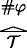is a grounding-tree $$\mathcal {T}$$ over (N,(S β ) β<α ) with # φ as its root, then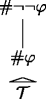is a grounding-tree over (N,(S β ) β<α ) with #¬¬φ as its root;

3. (iii)

ifis a grounding-tree $$\mathcal {T}$$ over (N, (S β ) β<α ) with # φ as its root, then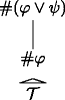is a grounding-tree over (N,(S β ) β<α ) with #(φψ) as its root;

4. (iv)

ifis a grounding-tree $$\mathcal {T}$$ over (N, (S β ) β<α ) with # ψ as its root, then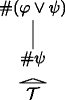is a grounding-tree over (N,(S β ) β<α ) with #(φψ) as its root;

5. (v)

ifare grounding-trees $$\mathcal {T}_{1},\mathcal {T}_{2}$$ over (N, (S β ) β<α ) with # φ,# ψ as their roots respectively, then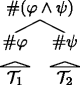is a grounding-tree over (N,(S β ) β<α ) with #(φψ) as its root;

6. (vi)

if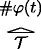is a grounding-tree $$\mathcal {T}$$ over (N, (S β ) β<α ) with # φ(t) as its root, then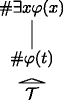is a grounding-tree over (N,(S β ) β<α ) with #x φ(x) as its root;

7. (vii)

if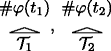, … are grounding-trees $$\mathcal {T}_{1},\mathcal {T}_{2}, \mathellipsis$$ over (N,(S β ) β<α ) with # φ(t 1),# φ(t 2),… as their roots respectively, where t 1,t 2,… are all and only the terms of $$\mathcal {L}_{PA}$$, then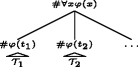is a grounding-tree over (N,(S β ) β<α ) with #x φ(x) as its root;

8. (viii)

ifis a grounding-tree $$\mathcal {T}$$ over (N, (S β ) β<α ) with #¬φ as its root, then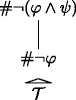is a grounding-tree over (N,(S β ) β<α ) with #¬(φψ) as its root;

9. (ix)

if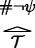is a grounding-tree $$\mathcal {T}$$ over (N, (S β ) β<α ) with #¬ψ as its root, then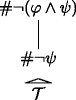is a grounding-tree over (N,(S β ) β<α ) with #¬(φψ) as its root;

10. (x)

if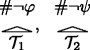are grounding-trees $$\mathcal {T}_{1},\mathcal {T}_{2}$$ over (N,(S β ) β<α ) with #¬φ,#¬ψ as their roots respectively, then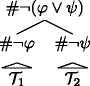is a grounding-tree over (N, (S β ) β<α ) with #¬(φψ) as its root;

11. (xi)

if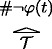is a grounding-tree $$\mathcal {T}$$ over (N, (S β ) β<α ) with #¬φ(t) as its root, thenis a grounding-tree over (N,(S β ) β<α ) with #¬∀x φ(x) as its root;

12. (xii)

if, … are grounding-trees $$\mathcal {T}_{1},\mathcal {T}_{2}, \mathellipsis$$ over (N,(S β ) β<α ) with #¬φ(t 1),#¬φ(t 2),… as their roots respectively, where t 1,t 2,… are all and only the terms of $$\mathcal {L}_{PA}$$, thenis a grounding-tree over (N,(S β ) β<α ) with #x φ(x) as its root;

13. (xiii)

if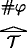is a grounding-tree $$\mathcal {T}$$ over (N,(S β ) β<α ) with # φ as its root and # φS β , for β < α, thenis a grounding-tree over (N,(S β ) β<α ) with $$\#Tr_{\beta }(\ulcorner \varphi \urcorner )$$ as its root;

14. (xiv)

if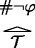is a grounding-tree $$\mathcal {T}$$ over (N,(S β ) β<α ) with #¬φ as its root and # φS β , for β < α, then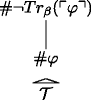is a grounding-tree over (N, (S β ) β<α ) with $$\#\neg Tr_{\beta }(\ulcorner \varphi \urcorner )$$ as its root;

15. (xv)

nothing else is a grounding-tree over (N,(S β ) β<α ).

Now, in contrast to grounding-trees over (N,S), grounding-trees over (N,(S β ) β<α ) can have an infinite height:

### Definition 6

We define the height $$h(\mathcal {T})$$ of a grounding tree over (N,(S β ) β<α ) by saying that:

1. (i)

all grounding-trees over (N,(S β ) β<α ) of the form # φS have height one;

2. (ii)

if $$\mathcal {T}$$ is a grounding-tree over (N,(S β ) β<α ) that is constructed from grounding-trees $$\mathcal {T}_{1}, \mathcal {T}_{2}, \mathellipsis$$ over (N,(S β ) β<α ), then the height of $$\mathcal {T}$$ is one plus the least upper bound of the heights of $$\mathcal {T}_{1}, \mathcal {T}_{2}, \mathellipsis$$:

$$h(\mathcal{T})=lub\{h(\mathcal{T}_{1}), h(\mathcal{T}_{2}), \mathellipsis\}+1,$$

where lub is the operation of taking the least upper bound.

We call a grounding-tree over (N,(S β ) β<α )degenerate iff it is of height one.

To see that there are grounding-trees of infinite height, let $$DN_{\overline {0}=\overline {0}}(x)$$ represent the property of being an instance of $$\overline {0}=\overline {0}$$ preceded by an even number of negations. Then it is easily checked that for all φ such that $$DN_{\overline {0}=\overline {0}}(\#\varphi ),$$ there is a grounding-tree of the form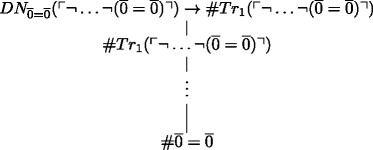which has height $$\frac {n}{2}+3,$$ where n is the number of negations in φ. A consequence of this is that the least upper bound of the heights of $$\mathcal {T}_{1}, \mathcal {T}_{2}, \mathellipsis$$ in the grounding-treeis at least ω and thus the height of this tree is at least ω + 1.Footnote 30

Now, an important consequence of this observation is that we can’t use ordinary induction on the height of trees to prove claims about all grounding-trees. We need to use transfinite induction on the height of the grounding-trees. This doesn’t add any further complications, but to be explicit let’s state the form of the principle that we’re going to use. Consider a property of grounding-trees. Then, if we can show that any degenerate grounding-tree has the property and we can show that if we can show that assuming that all trees of a height smaller than a given tree have the property, then the tree itself has the property, it follows that all grounding-trees have the property. Note that in this form of the principle, the induction step also includes limit cases, where we consider a tree of the height of a limit ordinal and need to show that the tree has the property in question, given that all trees of a lower height have the property.

Analogously to the case of grounding-trees over (N,S), we can now show that grounding-trees over (N,(S β ) β<α ) are: (i) rooted graphs over $$\bigcup _{\beta <\alpha }\mathbf {S}_{\beta }$$; (ii) indeed rooted trees over $$\bigcup _{\beta <\alpha }\mathbf {S}_{\beta }$$, i.e. they don’t contain any cycles; and finally, (iii) transitive.

### Lemma 6

Let 1 ≤ α𝜖 0, (N,(S β ) β<α )be the standard model of P R T <α ,and let $$\mathcal {T}$$ be a grounding-tree over (N,(S β ) β<α ). Then for all formulas $$\varphi \in \mathcal {L}_{<\alpha },$$ if # φ is a vertex in $$\mathcal {T},$$ then $$\#\varphi \in \bigcup _{\beta <\alpha }\mathbf {S}_{\beta }$$ .

### Proof

The new cases for clauses (xiii) and (xiv) follow by the fact that (N,(S β ) β<α ) is a model of P R T <α . □

Remember the notion of a code of a formula occurring below another code in a grounding-tree over (N,S). We now adapt this notion to grounding-trees over (N,(S β ) β<α ) by recursively saying that, for all 1 ≤ α𝜖 0, no code of any formula occurs below the code of any other formula in a degenerate grounding-tree over (N,(S β ) β<α ), and if $$\mathcal {T}$$ is a grounding-tree over (N,(S β ) β<α ) that was constructed from grounding-trees $$\mathcal {T}_{1}, \mathcal {T}_{2},\mathellipsis$$ over (N,(S β ) β<α ) according to the rules (ii–xvi) of Definition 5, then all occurrences of all formulas in $$\mathcal {T}_{1}, \mathcal {T}_{2},\mathellipsis$$ occur below the root of $$\mathcal {T}$$ in $$\mathcal {T}$$.

Then we can show:

### Lemma 7

Let 1 ≤ α𝜖 0 and let (N,(S β ) β<α )be the standard model of P R T <α . If $$\mathcal {T}$$ is a grounding-tree over (N,(S β ) β<α )with # φ as its root, for some formula $$\varphi \in \mathcal {L}_{<\alpha }$$ . Then, all formulas $$\psi \in \mathcal {L}_{<\alpha }$$ whose code # ψ occurs below # φ in $$\mathcal {T}$$ have a lower ω -complexity than φ.

### Lemma 8

Let 1 ≤ α𝜖 0, (N,(S β ) β<α )be the standard model of P R T <α ,and let $$\mathcal {T}$$ be a grounding-tree over (N,(S β ) β<α ). Then between any two nodes # φ and # ψ in $$\mathcal {T},$$ for formulas $$\varphi ,\psi \in \mathcal {L}_{<\alpha },$$ there is exactly one path.

### Lemma 9

Let 1 ≤ α𝜖 0 and let (N,(S β ) β<α )be the standard model of P R T <α . If there is a grounding-tree $$\mathcal {T}_{1}$$ over (N,(S β ) β<α )with # ψ as its root and # φ 1, # φ 2,…as its leaves and there is grounding-tree $$\mathcal {T}_{2}$$ over (N,(S β ) β<α )with # ψ, # ψ 1, # ψ 2,…as its leaves and # 𝜃 as its root, then there is a grounding-tree $$\mathcal {T}_{3}$$ over (N,(S β ) β<α )with # φ 1, # φ 2,…,# ψ 1, # ψ 2,…as its leaves and # 𝜃 as its root.

Finally, we define the standard model of P G A <α by saying that:

### Definition 7

Let 1 ≤ α𝜖 0 and let (N,(S β ) β<α ) be the standard model of P R T <α . We define the relation $$\mathbf {R}\subseteq \mathbb {N}^{2}$$ by saying that for all $$n,m\in \mathbb {N}$$, R(m,n) iff there is a non-degenerate grounding-tree over (N,(S β ) β<α ) with n as a leaf and m as its root.

Putting Lemmas 6, 8, and 9 together, we obtain:

### Theorem 2

(N,(S β ) β<α ,R)is a model of P G A <α ,for 1 ≤ α𝜖 0 , i.e. $$(\mathbf {N}, (\mathbf {S}_{\beta })_{\beta <\alpha }, \mathbf {R})\vDash PGA_{<\alpha }$$

### Proof

By Lemmas 6, 8, and 9, grounding-trees over (N,(S β ) β<α ) behave appropriately and satisfy the basic ground axioms. Since (N,(S β ) β<α ) is a model of P R T <α , the typed truth axioms are satisfied. The only new cases are the axioms for the typed Aristotelian principles. Here we only show that $$APU_{T}^{\gamma },$$ for γ < α holds:

• $$(\mathbf {N}, (\mathbf {S}_{\beta })_{\beta <\alpha }, \mathbf {R})\vDash \forall x (Tr_{\gamma }(x)\to x\lhd \underset {.}{Tr_{\gamma }}\dot {x})$$ for all γ < α.

Let σ be a variable assignment over (N,(S β ) β<α ,R) and $${\sigma ^{\prime }}$$ some x-variant of σ. Assume that $$(\mathbf {N}, (\mathbf {S}_{\beta })_{\beta <\alpha }, \mathbf {R})\vDash _{\sigma ^{\prime }} Tr_{\gamma }(x)$$. This means that $${\sigma ^{\prime }}(x)\in \mathbf {S}_{\gamma }$$. Since $$\mathbf {S}_{\gamma }=\{\#\varphi ~|~\varphi \in \mathcal {L}_{<\gamma },(\mathbf {N},(\mathbf {S}_{\delta })_{\delta <\gamma })\vDash \varphi \},$$ we know that $${\sigma ^{\prime }}(x)=\#\varphi ,$$ for some formula $$\varphi \in \mathcal {L}_{<\gamma }$$. Now, # φ is a degenerate grounding-tree over (N,(S β ) β<α ). But then, by clause (xiii) of Definition 5,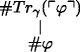is a non-degenerate grounding-tree over (N,(S β ) β<α ). Moreover, the root of this tree is $$\#Tr_{\gamma }(\ulcorner \varphi \urcorner )$$ and its only leaf is # φ. Now consider $${\sigma ^{\prime }}(\underset {.}{Tr_{\gamma }}\dot {x})$$. Since we know that $${\sigma ^{\prime }}(x)=\#\varphi$$ and $$\underset {.}{Tr_{\gamma }}$$ expresses the function that maps codes of formulas to the code of T r γ applied to the formula, we know that $${\sigma ^{\prime }}(\underset {.}{Tr_{\gamma }}\dot {\ulcorner \varphi \urcorner })=\#Tr_{\gamma }(\ulcorner \varphi \urcorner )$$. Thus, $$\mathbf {R}({\sigma ^{\prime }}(x), {\sigma ^{\prime }}(\underset {.}{Tr_{\gamma }}\dot {x}))$$ meaning $$\vDash _{\sigma ^{\prime }} x\lhd \underset {.}{Tr_{\gamma }}\dot {x}$$. And since σ was arbitrary, we get $$(\mathbf {N}, (\mathbf {S}_{\beta })_{\beta <\alpha }, \mathbf {R})\vDash \forall x (Tr_{\gamma }(x)\to x\lhd \underset {.}{Tr_{\gamma }}\dot {x}),$$ as desired.

We can show analogously that the other axioms hold. □

In the setting of PG (as well as P G A <α for 0 < α < 𝜖 0), we can accommodate new n-ary predicates R by stipulating:

$$\forall t_{1},\mathellipsis, \forall t_{n}(Tr(\d{\textit{R}}(t_{1}, \mathellipsis, t_{n}))\leftrightarrow R(t_{1}^{\circ},\mathellipsis, t_{n}^{\circ}))~\text{and}$$
$$\forall t_{1},\mathellipsis, \forall t_{n}(Tr(\underset{.}{\neg}\d{\textit{R}}(t_{1}, \mathellipsis, t_{n}))\leftrightarrow \neg R(t_{1}^{\circ},\mathellipsis, t_{n}^{\circ})).$$

If we furthermore add a theory for the new predicate R to our background theory, the resulting theory will be a theory of partial ground over the hierarchy of truths of that extended background theory. For example, we could formulate theories of partial ground over the truths of analysis, of mereology, or even of set-theory.Footnote 31 The resulting theory will then, of course, also prove all the instances of the T-scheme $$Tr(\ulcorner \varphi \urcorner )\leftrightarrow \varphi$$ over sentences of the new language $$\mathcal {L}\cup \{R\}$$. But this approach has limits. Of course, we can’t let R be our unary truth predicate T r itself. It is well-known that if we affirm:

$$\forall t(Tr(\underset{.}{Tr}(t))\leftrightarrow Tr(t^{\circ}))~\text{and}$$
$$\forall t(Tr(\underset{.}{\neg}\underset{.}{Tr}(t))\leftrightarrow \neg Tr(t^{\circ})),$$

our theory will fall prey to the liar paradox and its ilk. It might be somewhat surprising to learn, however, that we also can’t affirm:

$$\forall s\forall t(Tr(s\underset{.}{\lhd}t)\leftrightarrow s^{\circ}\lhd t^{\circ})~\text{and}$$
$$\forall s\forall t(Tr(s\underset{.}{\ntriangleleft}t)\leftrightarrow s^{\circ}\ntriangleleft t^{\circ})$$

in the context of predicational theories of ground. This will be the main result of this section.Footnote 32 We’ll call the resulting problem the new puzzle of ground.

Let’s move to a setting where we can affirm applications of the ground predicate to sentences involving the ground predicate. For this purpose, we have to make a couple of adjustments to our theory setup. In the following, let $$\mathcal {L}_{\lhd }$$ be the language $$\mathcal {L}\cup \{\lhd \}$$. Now first, we have to assume that we work in the context of a proper coding for $$\mathcal {L}_{\lhd }$$. In particular, we now assume that we have a name $$\ulcorner \varphi \urcorner$$ for every sentence $$\varphi \in \mathcal {L}_{\lhd }$$. We assume that we have a function symbol $$\underset {.}{\lhd }$$ such that:

$$\vdash_{PA} s\underset{.}{\lhd}t=\ulcorner s\lhd t\urcorner,$$

for all terms s and t. And we let $$Sent_{\lhd }$$ abbreviate the formula that allows us to represent the set (of codes of) sentences of $$\mathcal {L}_{\lhd }$$. In particular, we assume that for all $$n\in \mathbb {N}$$:

$$\begin{array}{@{}rcl@{}} \vdash_{PA} Sent_{\lhd}(\overline{n}) \text{ iff } n\in \#\mathcal{L}_{\lhd} \text{ and }\\ \vdash_{PA} \neg Sent_{\lhd}(\overline{n}) \text{ iff } n\not\in \#\mathcal{L}_{\lhd}. \end{array}$$

Second, we need to adjust the axiom T 3 to:

$$(T_3^{\lhd}) \forall x(Tr(x)\to Sent_{\lhd}(x)),$$

which allows sentences involving the ground predicate to occur in the context of the truth predicate (and thus in the context of the ground predicate). We arrive at a modified predicational theory of ground:

### Definition 8

The predicational theory PUG of untyped ground consists of the axioms of PG without the axiom T 3, plus the axiom $$T_3^{\lhd }$$ and the axioms:

$$(T_{\lhd}^{1}) \quad \forall s\forall t(Tr(s\underset{.}{\lhd}t)\leftrightarrow s^{\circ}\lhd t^{\circ})~\text{and}$$
$$(T_{\lhd}^{2}) \quad \forall s\forall t(Tr(s\underset{.}{\ntriangleleft}t)\leftrightarrow s^{\circ}\ntriangleleft t^{\circ}).$$

We will now show that PUG is inconsistent. To see this, first note that we can show in the same way as in the case of PG that PUG proves the uniform T-scheme for sentences involving the ground predicate:

### Lemma 10

For all sentences $$\varphi \in \mathcal {L}_{\lhd },$$

$$\vdash_{PUG} \forall t_{1}, \mathellipsis,\forall t_{n}(Tr(\varphi(\underset{.}{t_{1}}, \mathellipsis, \underset{.}{t_{n}}))\leftrightarrow \varphi(t_{1}^{\circ},\mathellipsis, t_{n}^{\circ})).$$

### Proof

By induction on the positive complexity of formulas. The new axioms $$T_{\lhd }^{1/2}$$ take care of the new base-case. □

Note that this lemma doesn’t entail yet that PUG is inconsistent: the truth predicate T r is not in the language $$\mathcal {L}_{\lhd }$$ and thus Lemma 10 doesn’t entail that we’re applying the truth predicate to sentences involving the same truth predicate. To see that PUG is inconsistent, we need to do some more work. Since we work in the context of a coding for $$\mathcal {L}_{\lhd },$$ we can get the diagonal lemma for the language:

### Lemma 11

For all formulas $$\varphi \in \mathcal {L}_{\lhd }$$ with exactly one free variable, there is a formula $$\delta \in \mathcal {L}_{\lhd }$$ such that

$$\vdash_{PA} \delta\leftrightarrow \varphi(\ulcorner\delta\urcorner).$$

With these two lemmas in place, we can show our main result:

### Theorem 3

PUG is inconsistent.

### Proof

Let φ(x) be the formula $$\neg (x\lhd \underset {.}{\neg }\underset {.}{\neg }x)$$. By the diagonal lemma for $$\mathcal {L}_{\lhd }$$, we know that there is a formula $$\delta \in \mathcal {L}_{\lhd }$$ such that:

$$\vdash_{PA}\delta\leftrightarrow\neg(\ulcorner\delta\urcorner\lhd \ulcorner\neg\neg\delta\urcorner).$$

It is easily checked that we can now both prove δ and ¬δ. □

We are left with yet another puzzle of ground: by letting the truth predicate (and thus the ground predicate) apply to truths involving the ground predicate, we made our intuitively plausible theory of ground inconsistent. But intuitively, we want to be able to talk about the truth of sentences involving the ground predicate. So what went wrong?

First, note that the new puzzle is different from Fine’s puzzle of ground. Fine’s puzzle consists in the fact that different intuitively plausible principles for partial ground and truth entail that the truth of some sentences partially ground themselves—in contradiction to the irreflexivity of partial ground. Our puzzle, in contrast, consists in the fact that letting the truth predicate apply to the ground predicate makes our previously consistent principles of ground inconsistent.

Moreover, note that the use of double negation (and of the corresponding upward directed ground axiom) in the proof of Theorem 3 is dispensable. We could equally well have applied the diagonal lemma to the formula $$\neg (x\lhd x\underset {.}{\lor }x)$$ or $$\neg (x\lhd x\underset {.}{\land }x)$$ or … and we would still have gotten the same inconsistency result (using the corresponding upward directed ground axioms for these connectives). The point is that our paradox is not a paradox of double negation, or disjunction, or conjunction, or the like—it has another source.

We argue that the paradox is a paradox of self-reference in the context of partial ground. In support of this claim, first note that all the different sentences that we could use for the proof of Theorem 3 have in common that they (provably) “say of themselves” that they violate certain principles of partial ground, and as a consequence they are inconsistent over our theory of untyped ground. Thus, the new puzzle of ground bears a strong resemblance to the classic semantic paradoxes. Indeed, we can make this analogy even more explicit in the following proposition. Let’s define the predicate T r0= by the following explicit definition:

$$\forall x(Tr_{0}^=(x)\leftrightarrow_{def} \exists s,t(x=(s\underset{.}{=} t)\land s^{\circ}= t^{\circ})\lor \exists s,t(x=(s\underset{.}{\neq} t)\land s^{\circ}\neq t^{\circ}))$$

And let’s define the predicate $$Tr_0^{\lhd }$$ by the following explicit definition:

$$\forall x(Tr_{0}^{\lhd}(x)\leftrightarrow_{def} \exists s,t(x=(s\underset{.}{\lhd} t)\land s^{\circ}\lhd t^{\circ})\lor \exists s,t(x=(s\underset{.}{\ntriangleleft} t)\land s^{\circ}\ntriangleleft t^{\circ}))$$

So, intuitively T r0= and $$Tr_0^{\lhd }$$ are truth predicates for the literals of $$\mathcal {L}_{\lhd }$$. We can then show the following proposition:

### Proposition 2

PUG proves that the formula $$Tr_0^=(x)\lor Tr_0^{\lhd }(x)\lor \exists y(y\lhd x)$$ satisfies the T-scheme for the formulas of $$\mathcal {L}_{\lhd },$$ i.e. for all $$\varphi \in \mathcal {L}_{\lhd }$$ :

$$\vdash_{PUG} Tr_{0}^=(\ulcorner\varphi\urcorner)\lor Tr_{0}^{\lhd}(\ulcorner\varphi\urcorner)\lor \exists y(y\lhd \ulcorner\varphi\urcorner)\leftrightarrow \varphi.$$

### Proof

By induction on the positive complexity of φ for both directions of the biconditional. The base cases for φ being s = t or st, for terms s and t, are covered by the basic truth axioms T 1 and T 2 and the definition of T r0=. Similarly, the base cases for φ being $$s\lhd t$$ or $$s\ntriangleleft t,$$ for terms s and t, are covered by the axioms $$T_{\lhd }^1$$ and $$T_{\lhd }^2$$ and the definition of $$Tr_0^{\lhd }$$. The remaining cases are can be dealt with using Lemma 10. □

In other words, in PUG we can define a truth predicate for $$\mathcal {L}_{\lhd }$$ that satisfies the T-scheme for $$\mathcal {L}_{\lhd }$$. It follows by Tarski’s theorem of the undefinability of truth that PUG is inconsistent . Our Theorem 3 is only a special case of this more general fact, as it were. This is the precise sense in which the ground predicate “behaves too much like a truth predicate”—in other words: the new puzzle of ground is at heart a paradox of self-reference.

## Conclusion

In this paper, we have provided a typed solution to the puzzle of ground. But there are a couple of worries that should be addressed: First, we might be worried that we used “a sledgehammer to crack a walnut:” our use of typing up to 𝜖 0 could be seen as a slight overkill to solve such a simple problem as the puzzle of ground. We would like to say two things in response to this worry: First, the use of typing up to 𝜖 0 is not really necessary to formulate the solution. We could already have stopped at level two, where in the typed setting grounding between arithmetic truths and truths involving a truth predicate occurs for the first time: it follows from our results that the theory P G A 2 is consistent and proves the following simple version of the typed Aristotelian principle for truth:

$$\forall x(Tr_{1}(x)\to x\lhd \underset{.}{Tr_{1}}(\dot{x})).$$

But there is no real reason to stop at level two. We have shown that the methods of the paper work up to the level 𝜖 0. Moreover, the result was an intuitive and mathematically non-trivial theory of partial ground and typed truth. Thus, the results of this paper support our plea for collaboration between ground-theorists and truth-theorists.

Second, there is the worry that by “going typed” we have “thrown out the baby with the bathwater:” There are several unproblematic self-referential sentences that fall out of the scope of our typed theory. For example, by a simple application of the diagonal lemma to the formula T r(x), we get the truth-teller sentence ρ such that:

$$\vdash_{PA} \rho\leftrightarrow Tr(\ulcorner\rho\urcorner).$$

This sentence can consistently be added to a theory that proves the instance of the T-scheme for it. It might be argued that this shows that we should include it in our best theory of partial ground and truth. PA and the T-scheme don’t decide this sentence: we can both consistently affirm it and its negation (although, of course, not at the same time). Thus, it might be argued, a good theory of partial ground and truth should at least prove the following principle:

$$\rho\to \ulcorner\rho\urcorner\lhd \ulcorner Tr(\ulcorner\rho\urcorner)\urcorner.$$

This sentence, however, is in none of the languages $$\mathcal {L}_{<\alpha }^{\lhd }$$, for 0 < α < 𝜖 0, and so in these languages we cannot say anything about its partial grounds. To make things worse, it could be argued that the principles of partial ground and truth should even apply to the liar sentence λ such that $$\vdash _{PA}\lambda \leftrightarrow \neg Tr(\ulcorner \lambda \urcorner )$$. In particular, it might be argued that both

$$\lambda \to \ulcorner \lambda \urcorner \lhd \ulcorner \neg Tr(\ulcorner \lambda \urcorner )\urcorner$$ and $$\neg \lambda \to \ulcorner \neg \lambda \urcorner \lhd \ulcorner Tr(\ulcorner \lambda \urcorner )\urcorner$$ should be the provable. The argument would be that assuming that if λ is true, then since λ “says of itself” that it is not true, the truth of λ grounds the truth of $$\neg Tr(\ulcorner \lambda \urcorner )$$ and analogously for ¬λ. In the context of the factivity of ground, this would lead to a non-classical theory of truth. If we have:

$$\forall x\forall y(x\lhd y\to Tr(x)\land Tr(y)),$$

then we would immediately get $$Tr(\ulcorner \lambda \urcorner )$$ and $$Tr(\ulcorner \neg \lambda \urcorner )$$ from the above principles. But there are non-classical theories of truth on the market, where this would indeed be plausible—so what’s the problem?

So far, we have axiomatized a classical theory of partial ground and truth, and we have achieved this by typing our truth predicate. We could also try to axiomatize non-classical theories of partial ground and truth, for example in the spirit of the Kripke-Feferman theory of truth KF [8, p. 195–227]. In such a setting we could consistently affirm the existence of the liar sentence and the truth-teller sentence, and even apply the principles of partial ground to them—which is, of course, ruled out on our approach. We would get a theory of partial ground and untyped truth. But such a project would require the development of new methods and this goes beyond the scope of this paper. Most importantly, in such a setting, we would have to find a new solution to the puzzle of ground, since we relied on the typing of the truth predicate for our solution. In the terminology of [6, p. 110], our solution is a predicativist solution to the puzzle of ground: On our approach, the grounds of a truth of the form ∃x T r α (x) never includes truths of involving a truth predicate T r α , where α is some ordinal with 0 < α < 𝜖 0—in particular, the grounds of the truth of ∃x T r α (x) don’t include the truth of ∃x T r α (x) itself. We have shown that by respecting the typing restrictions of Tarski’s hierarchy, we can obtain a consistent theory of partial ground that proves (typed versions of) the Aristotelian principles. There is, of course, room for impredicativist solutions in the terminology of [6, p. 110], but developing such solutions is beyond the scope of this paper. We leave this for future research.

How should we respond to the paradox of self-referential ground? Three natural ways in which we could try to block the inconsistency theorem suggest themselves: First, we could try to rule out self-referential sentences of ground like the one used in the proof of the inconsistency theorem. Second, we could try to restrict the principles of partial ground used in the proof of the inconsistency theorem. And third, we could try to formulate a non-standard logic of ground that does not sanction the logical principles used in the proof of the inconsistency theorem. The analogy between our inconsistency theorem (Theorem 3) and the Tarski’s theorem of the undefinability of truth (Proposition 2) suggests a neat terminology for these approaches. Analogously to theories of truth , we get: typed theories of partial ground, which avoid paradox by putting type-restrictions on the relation of partial ground, effectively ruling out self-referential sentences like the one in the proof; untyped theories of partial ground, which avoid paradox by restricting the principles of partial ground; and finally non-classical theories of partial ground, which avoid paradox (or: triviality) by abandoning classical logic in favor of alternative logics.

The results of this paper all point in the direction of a typed theory of partial ground. Indeed our theories P G A <α are typed theories of partial ground: the axiom $$G_4^{\alpha }$$ is effectively a typing axiom. Moreover, we have shown that the theories P G A <α , for 1 ≤ α𝜖 0, are consistent (Corollary 1). Thus, there is good evidence that a typed solution to the new puzzle of ground works. A natural direction to take from here would be to extend Tarski’s truth-theoretic hierarchy to a truth- and ground-theoretic hierarchy. We would type the ground predicate to get a family of predicates: $$\lhd _1, \lhd _2, \mathellipsis$$. We would end up with a doubly-typed theory: typed with respect to truth and typed with respect to ground. The results of this paper suggest that this theory will turn out consistent and this provides further support for the claim that we should use typing in the context of theories of ground. But carrying out the details of this proposal is beyond the scope of this paper. Also this we leave for future research.Footnote 33

1. For part one, see  in this journal.

2. For a more comprehensive introduction to the concept of (partial) ground we rely on in this paper and further literature references, see Sections 1 and 2 of the first part of this paper.

3. This is, in any case, the standard view of partial ground. Some authors have challenged the view, though:  challenges the claim that partial ground is irreflexive and  challenges the claim that partial ground is transitive. See  and  for a defense of the standard view against these challenges.

4. For a precis of the first part of the paper, see Section 1.1 below.

5. Here we use T r as our truth-predicate and the Gödel-corners $$\ulcorner \phantom {\varphi }\urcorner$$ as our quotation device. For more on these syntactic matters, see Section 1.1 below.

6. For a detailed exposition of typed theories of truth, see [8, p. 49–286].

7. Stephan Krämer  and Thomas Donaldson  tentatively sketch predicativist solutions without developing the formal details. Fine , Correia , and Litland  propose non-predicativist solutions to the puzzle of ground. While Correia gives up the irreflexivity of ground, Litland and Fine do not.

8. This generalizes some previously results obtained by the author in .

9. For a more detailed account of the syntax theory employed throughout the paper, see Section 3 of the first part of this paper or [8, p. 29–35].

10. In formulating these axioms, we make use of the function symbol to represent the valuation function val in the standard model, i.e. s = t means that the denotation of s is t. Officially, however, this is merely an abbreviation for the more complicated defining formula of the valuation function (which exists by standard representation results). Officially, we cannot have symbol representing the valuation function in our language, since then we’d run the risk of inconsistency. For more on this, compare [8, p. 32].

11. To illustrate how the quantified axioms work, consider a formula φ such that $$Tr(\ulcorner \varphi \urcorner )$$. Then the principle AP T says that $$\ulcorner \varphi \urcorner \lhd \underset {.}{Tr}\dot {\ulcorner \varphi \urcorner }$$. But the latter is $$\ulcorner \varphi \urcorner \lhd \ulcorner Tr(\ulcorner \varphi \urcorner )\urcorner ,$$ since $$\dot {\ulcorner \varphi \urcorner }=\ulcorner \ulcorner \varphi \urcorner \urcorner$$ and $$\underset {.}{Tr}(\ulcorner \ulcorner \varphi \urcorner \urcorner )=\ulcorner Tr(\ulcorner \varphi \urcorner )\urcorner$$. So AP T allows us to prove $$Tr(\ulcorner \varphi \urcorner )\to \ulcorner \varphi \urcorner \lhd \ulcorner Tr(\ulcorner \varphi \urcorner )\urcorner ,$$ for every sentence φ. The quantified principle AP F works analogously.

12. Fine works in an operational framework, but his argument can easily be adapted to the present framework. Fine’s argument is discussed and refined by [2, 14].

13. Fine, in his original paper , discusses a range of ground-theoretic puzzles that arise in a similar way from principles similar to the Aristotelian principle. Here we’ll focus on the puzzle about truth and partial ground, because the problem arises most naturally in the present context.

14. For a proof, see for example [1, p. 220–224].

15. Here and in the following, we will use a weak concept of self-reference, where a sentence that is (provably) equivalent to a sentence that involves its own Gödel numeral is said to be self-referential. In this sense, the diagonal lemma as stated above gives us a sentence that is self-referential. There is a stronger sense of self-reference, in which a sentence is only self-referential if it is identical to a sentence that involves it’s own Gödel numeral. There are stronger versions of the diagonal lemma that would give us self-reference in this sense as well, but for simplicity’s sake, we’ll stick to the weak concept of self-reference throughout the paper. For a more comprehensive discussion of these issues, see, e.g., .

16. However, as  shows there are paradoxes without self-reference. Moreover, there are self-referential sentences that are not paradoxical. But still, the intuitive view is that in the case of the liar and similar paradoxes, self-reference plays an essential role.

17. This language is then, of course, an extension of the language of PA: it extends the purely arithmetic vocabulary with names for the sentences of $$\mathcal {L}$$ and a truth predicate for those sentences.

18. This also applies to paradoxes without self-reference, such as Yablo’s paradox: Yablo similarly formulates his paradox in a semantically closed language.

19. There is also a kind of semantic self-reference involved in the case of puzzle. The existential quantifier in ∃x T r(x) semantically ranges over all sentences of $$\mathcal {L}_{Tr}$$, including ∃x T r(x) itself. Thus the truth of ∃x T r(x) is partially witnessed by the truth of ∃x T r(x). But here we do not wish to push this point any further.

20. For more on axiomatizations of Tarski’s hierarchy, see [8, p. 125–29].

21. We assume that the reader is familiar with the basic theory of ordinals. For the relevant definitions, see [10, p. 17–26], for example. The ordinal 𝜖 0 is the limit of the sequence $$1,\omega , \omega ^{\omega }, \omega ^{\omega ^{\omega }},\mathellipsis$$; in other words, 𝜖 0 is the first ordinal that satisfies the equation ω x = x. This ordinal 𝜖 0 is still countable, i.e. it has the same cardinality as the set of the natural numbers. But it provides a natural stopping point for our infinitary hierarchy, since (i) we can code the ordinals below 𝜖 0 and (ii) PA represents the well-ordering of the ordinals below 𝜖 0. We will not go into the details here, as the infinitary nature of our hierarchy is not particularly important to our philosophical point. Nevertheless, for reasons of generality, we will extend our hierarchy to this level, since 𝜖 0 is the limit up to where we can apply the methods of this paper.

22. Here it is important that we’re restricting ourselves to countable ordinals, because otherwise we would “run out of codes” at some point.

23. For the proof note that if we assume that T r 1(t) for a term t, it follows by axiom T$$_{1}^{1}$$ that S e n t <1(t) and thus we can prove in PA that $$Sent_{<2}(\underset {.}{Tr_{1}}(t))$$. Similarly, if we assume $$Tr_{2}(\underset {.}{Tr_{1}}\dot {t}),$$ we can prove that S e n t <1(t) by T$$^{2}_{3}$$ and PA.

24. We can formulate a slightly stronger version of P R T <α by replacing the schematic axioms $$RP_{12}^{\beta }$$ and $$RP_{13}^{\beta }$$ with the quantified axioms: $$\forall x\forall \gamma \underset {.}{<}\ulcorner \beta \urcorner (Sent_{<\gamma }(x)\to (Tr_{\beta }(\underset {.}{Tr_{\gamma }}\dot {x})\leftrightarrow Tr_{\beta }(x)))$$ and $$\forall x\forall \gamma \underset {.}{<}\ulcorner \beta \urcorner (Sent_{<\gamma }(x)\to (Tr_{\beta }(\underset {.}{\neg }\underset {.}{Tr_{\gamma }}\dot {x})\leftrightarrow Tr_{\beta }(\underset {.}{\neg }x)))$$, where ∀γ quantifies over codes of ordinals, $$\ulcorner \beta \urcorner$$ is a term for a code of the ordinal β, and $$\underset {.}{<}$$ represents the well-ordering on ordinals. To properly formulate these axioms, we require a coding of the ordinals up to 𝜖 0, a representation of the natural well-ordering of these ordinals, and a justification for quantifying into ordinal indexes in S e n t <γ and $$\underset {.}{Tr_{\gamma }}$$. We will discuss such a coding below, but for reasons of perspicuity, we will stick with the slightly weaker schematic version of P R T <α . Also, the (schematic) theory P R T <α is equivalent to the (schematic) theory R T <α of ramified truth up to α, which is the typed version of CT. As in the case of PT and C T, we will take the meta-theorems of R T <α and apply them immediately to P R T <α .

25. Now we could define quantification over ordinals by saying that ∀γ φ means $$\forall x(\underset {.}{On_{<\epsilon _{0}}}(x)\to \varphi )$$ and work with the more general versions of the axioms mentioned before. But for reasons of perspicuity, we refrain from doing so.

26. Here lub isthe operation of taking the least upper bound with respect to the standard partial ordering ≼on the ordinals.

27. We don't give the detailed proof here, but it essentially proceeds by using induction on ordinals below ϵ0 in PA.

28. We could also use the theorem to determine the proof theoretic strength of P G A α , but there is a small “hiccup:” the version of P R T α that we discussed here is not exactly the one that is usually discussed in the literature. As mentioned in Footnote 24, P R T α is usually formulated using quantification over ordinals, which we avoided here for reasons of perspicuity. The version of P R T α with axioms quantifying over ordinals proves the same arithmetical theorems as the theory R A α of ramified analysis up to (and including) α. For a proof of this result, see . We suspect that the proof theoretic strength of our version of P R T α is very close to this, although we’re not going to prove anything to this effect.

29. The situation is quite similar to the corresponding theories of truth. For a discussion of the natural stopping point see [8, p. 322-29].

30. In fact, by a slightly more complicated argument we can show that the height of this tree is exactly ω + 1.

31. The truths of new atomic sentences will, of course, not have any provable grounds in the theory.

32. Here $$s\ntriangleleft t,$$ for terms s and t, is an abbreviation of $$\neg (s\lhd t),$$ analogous to the case of st. Similarly, the notation $$s\underset {.}{\ntriangleleft }t$$ is an abbreviation for the complex function term $$\underset {.}{\neg }(s\underset {.}{\lhd }t)$$ for terms s and t.

33. A particularly interesting point that I’ll leave for future research has been brought to my attention by an anonymous referee: next to the kind of self-reference we obtain, for example, by means of the diagonal lemma, there is also what’s sometimes called empirical self-reference: self-reference that depends on contingent facts about the world. Consider the sentence “the last example sentence in the paper Axiomatic Theories of Partial Ground II. Partial Ground and Hierarchies of Typed Truth does not partially ground its own double negation,” for example. Properly formalized, using, for example, a description operator, this sentence will create a ground-theoretic paradox of self-reference in the same way as the sentence δ in the proof of Theorem 3—but only because it is in fact the last such example sentence, otherwise it would simply be false. To deal with such forms of self-reference in a typed theory, we would presumably need to introduce some kind of context dependent typing. But alas, working out the details goes beyond the scope of this paper.

## References

1. Boolos, G. et al. (2007). Computability and logic. Cambridge University Press.

2. Correia, F. (2013). Logical grounds, Review of Symbolic Logic 7.1 (pp. 31–59).

3. Donaldson, T. (2016). The (metaphysical) foundations of arithmetic?, Noûs. doi:10.1111/nous.12147.

4. Feferman, S. (1991). Reflecting on incompleteness. Journal of Symbolic Logic, 56.1, 1–49.

5. Fine, K. (2012). Guide to Ground. In Correia, F., & Schnieder, B. (Eds.), Metaphysical Grounding: Understanding the Structure of Reality (pp. 37–80): Cambridge University Press.

6. Fine, K. (2010). Some puzzles of ground, Notre Dame Journal of Formal Logic 51.1 (pp. 97–118).

7. Fine, K. (2012). The pure logic of ground, Review of Symbolic Logic 5.1 (pp. 1–25).

8. Halbach, V. (2011). Axiomatic theories of truth. Cambridge University Press.

9. Halbach, V., & Visser, A. (2014). Self-reference in arithmetic I, Review of Symbolic Logic 7.4 (pp. 671–691).

10. Jech, T. (2002). Set theory. Springer.

11. Jenkins, C.S. (2011). Is metaphysical dependence irreflexive?, The Monist 94.2 (pp. 267–276).

12. Korbmacher, J. (forthcoming). Axiomatic Theories of Partial Ground I, Journal of Philosophical Logic (pp. 1–31).

13. Korbmacher, J. (2015). Yet another puzzle of ground, Kriterion 29.2 (pp. 1–10).

14. Krämer, S. (2013). A simpler puzzle of ground, Thought: A Journal of Philosophy 2.2 (pp. 85–89).

15. Litland, J.E. (2015). Grounding, Explanation, and the limit of internality, Philosophical review 124.4.

16. Litland, J.E. (2013). On some counterexamples to the transitivity of grounding, Essays in Philosophy 14.1 (pp. 19–32).

17. Pohlers, W. (2009). Proof Theory. The first step into impredicativity. Springer.

18. Raven, M.J. (2013). Is ground a strict partial order?, American Philosophical Quarterly 50.2 (pp. 191–99).

19. Schaffer, J. (2012). Grounding, transitivity, and contrastivity. In Correia, F., & Schnieder, B. (Eds.), Metaphysical Grounding: Understanding the Structure of Reality (pp. 122–138): Cambridge University Press.

20. Tarski, A. (1944). The semantic conception of truth: and the foundations of semantics, Philosophy and Phenomenological Research 4.3 (pp. 341–76).

21. Yablo, S. (1993). Paradox Without Self-Reference, Analysis 53.4 (pp. 251–52).

## Acknowledgements

I’d like to thank Hannes Leitgeb, Benjamin Schnieder, Albert Anglberger, Thomas Schindler, Lavinia Picollo, Johannes Stern, Martin Fischer, Tim Button, the participants of the workshop “Logical and Metaphysical Perspectives on Grounding” at the GAP.9 in Osnabrück, O. Foisch, and two anonymous referees for their helpful comments and suggestions. Part of this research was supported by the Alexander von Humboldt Foundation.

## Author information

Authors

### Corresponding author

Correspondence to Johannes Korbmacher.